# RD Sharma Solutions for Class 8 Maths Chapter 17 Understanding Shapes- III (Special Types of Quadrilaterals) Exercise 17.1

In Exercise 17.1 of Chapter 17, we shall discuss problems based on various types of quadrilaterals like – trapezium, isosceles trapezium, parallelogram, rhombus, rectangle, square. In this exercise, we mainly study the properties of a parallelogram. Students who find difficulty in understanding the concepts can refer to RD Sharma Solutions which is prepared by our expert tutors with utmost care, which helps students gain competence on the concept. Ultimately the main aim is to help students boost their confidence level and achieve high marks in their exams. Pdf can be downloaded easily from the links given below.

## Download the Pdf of RD Sharma Solutions for Class 8 Maths Exercise 17.1 Chapter 17 Understanding Shapes- III (Special Types of Quadrilaterals)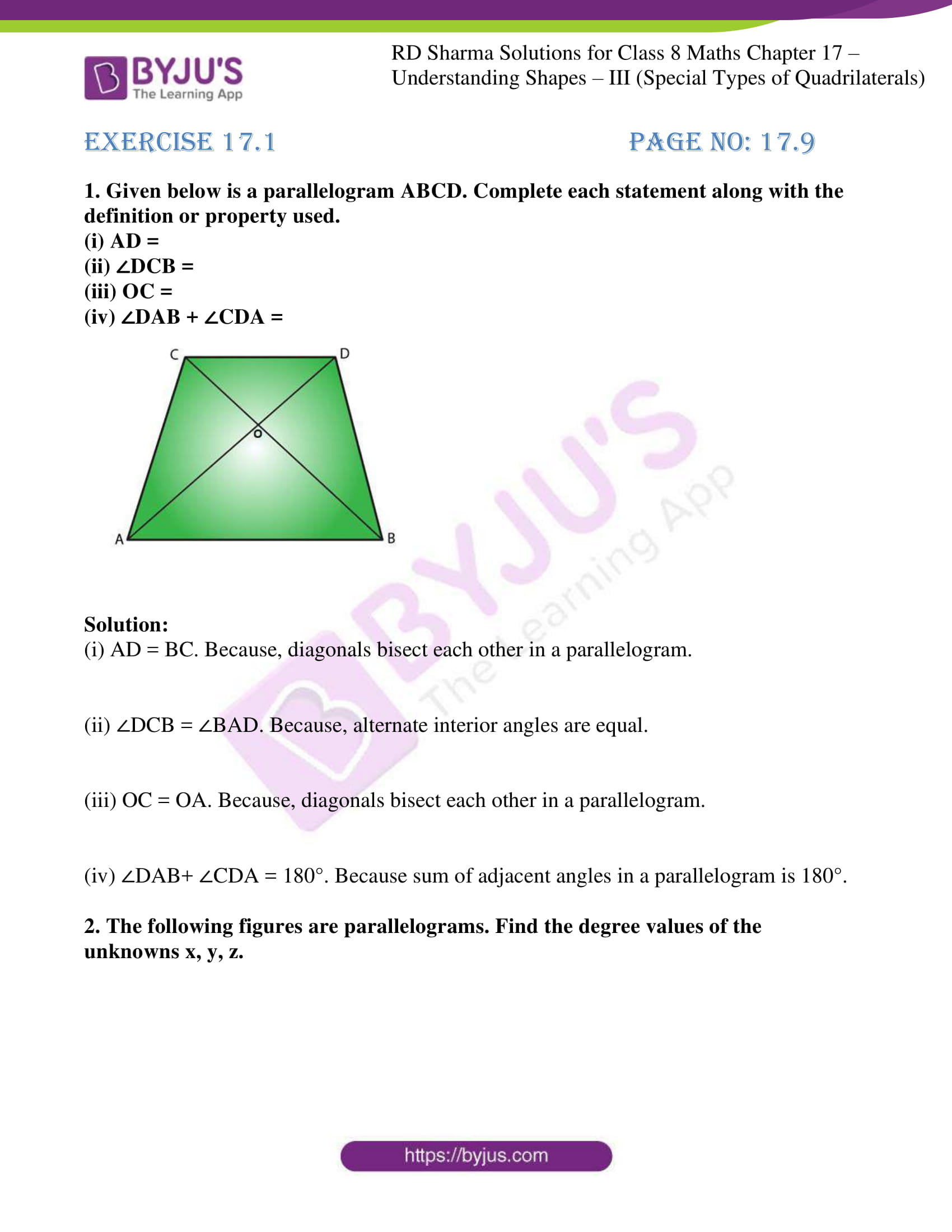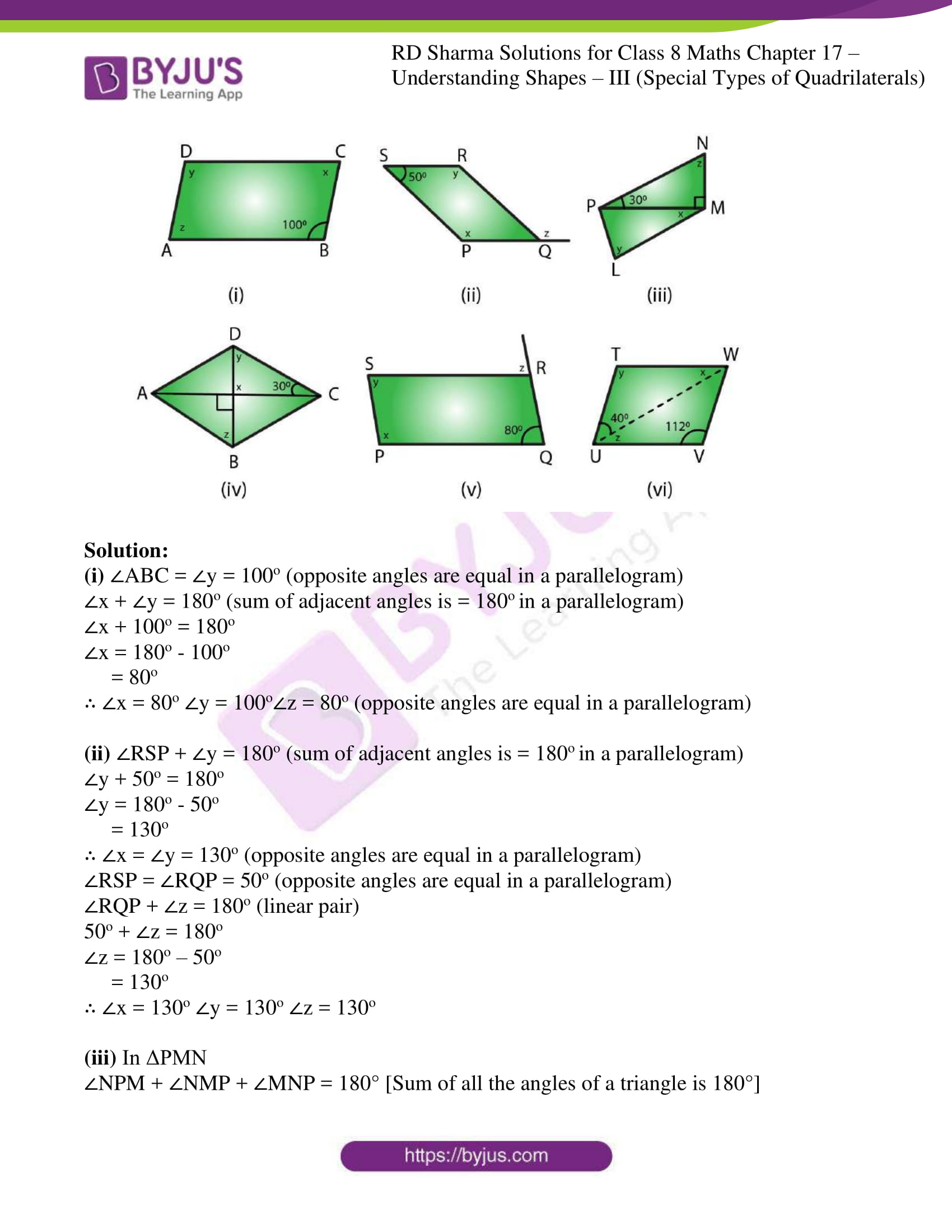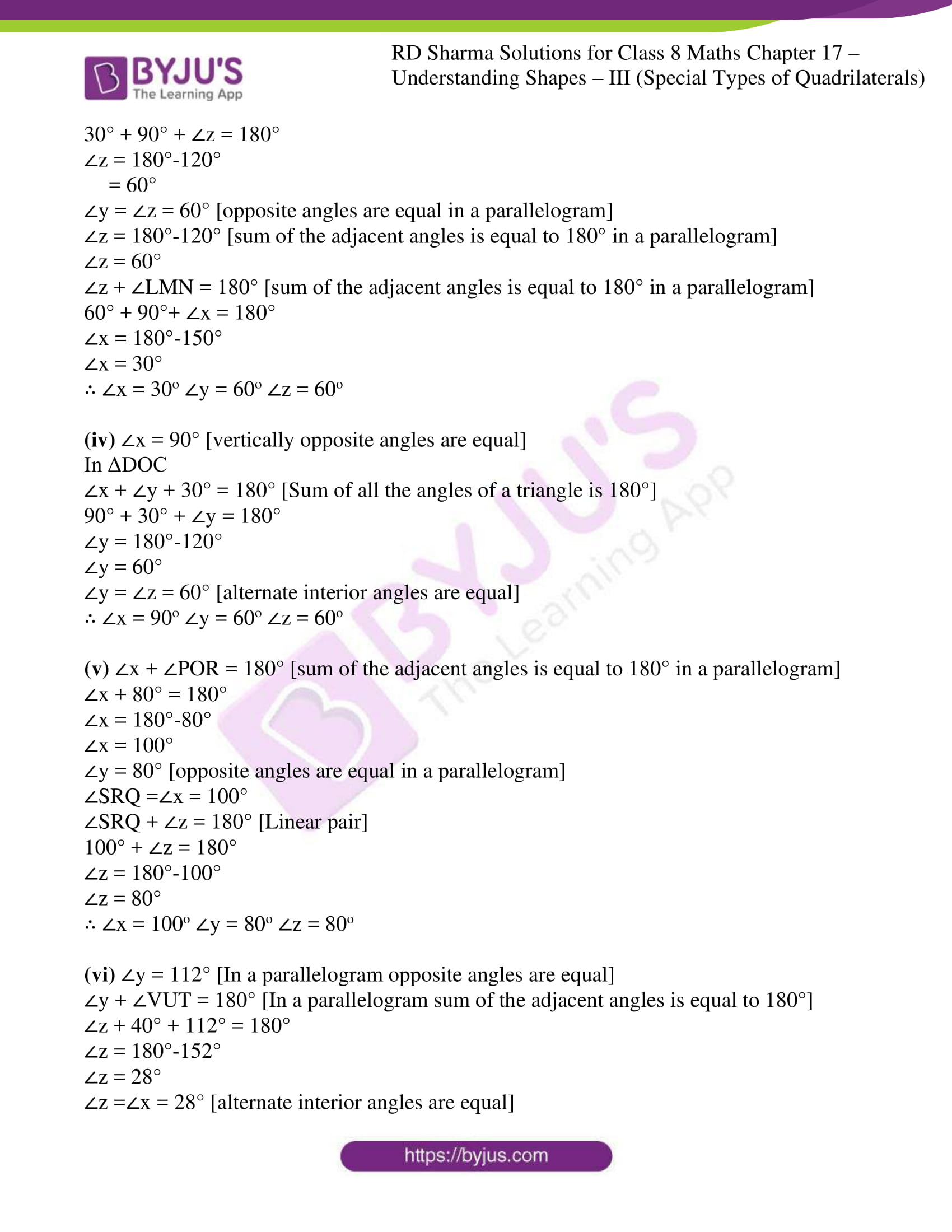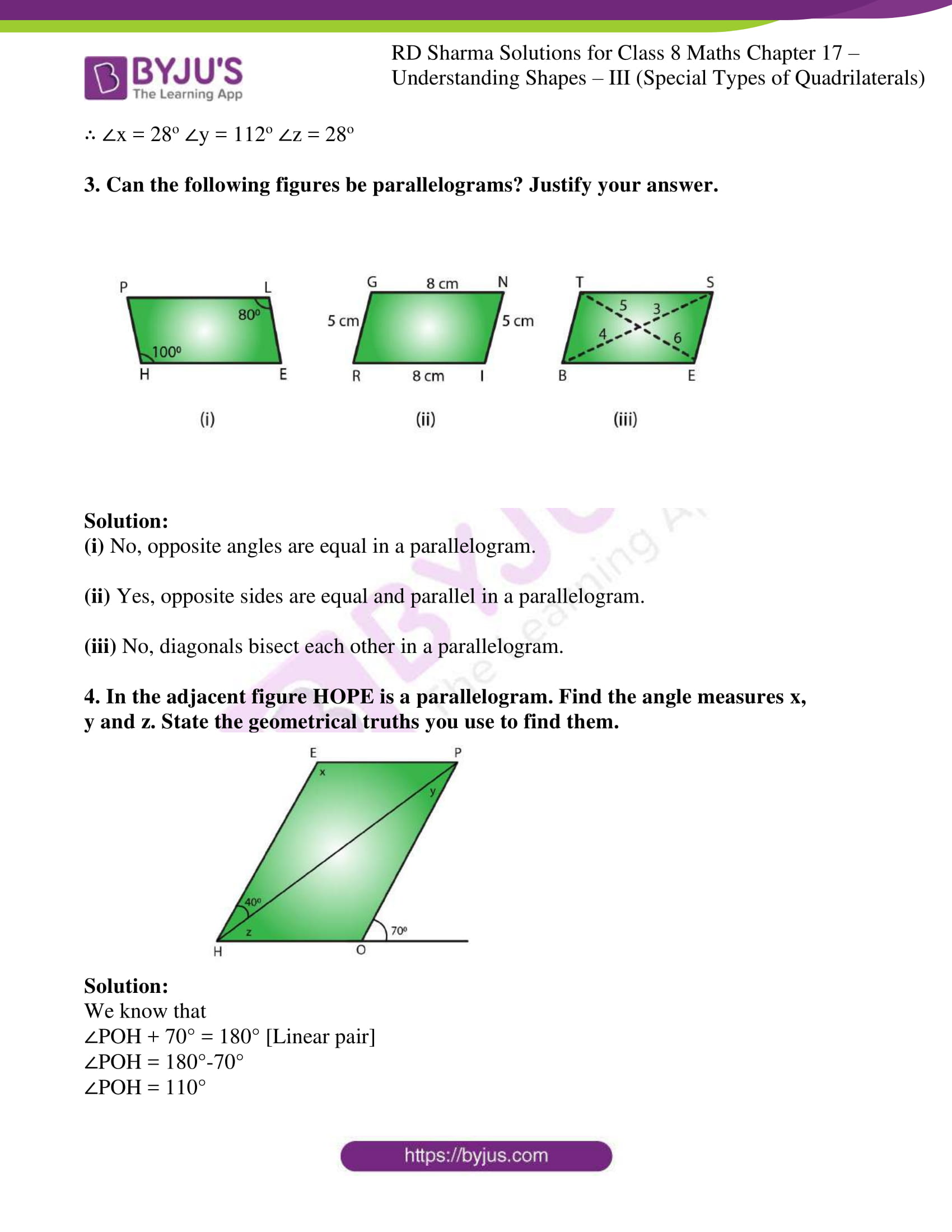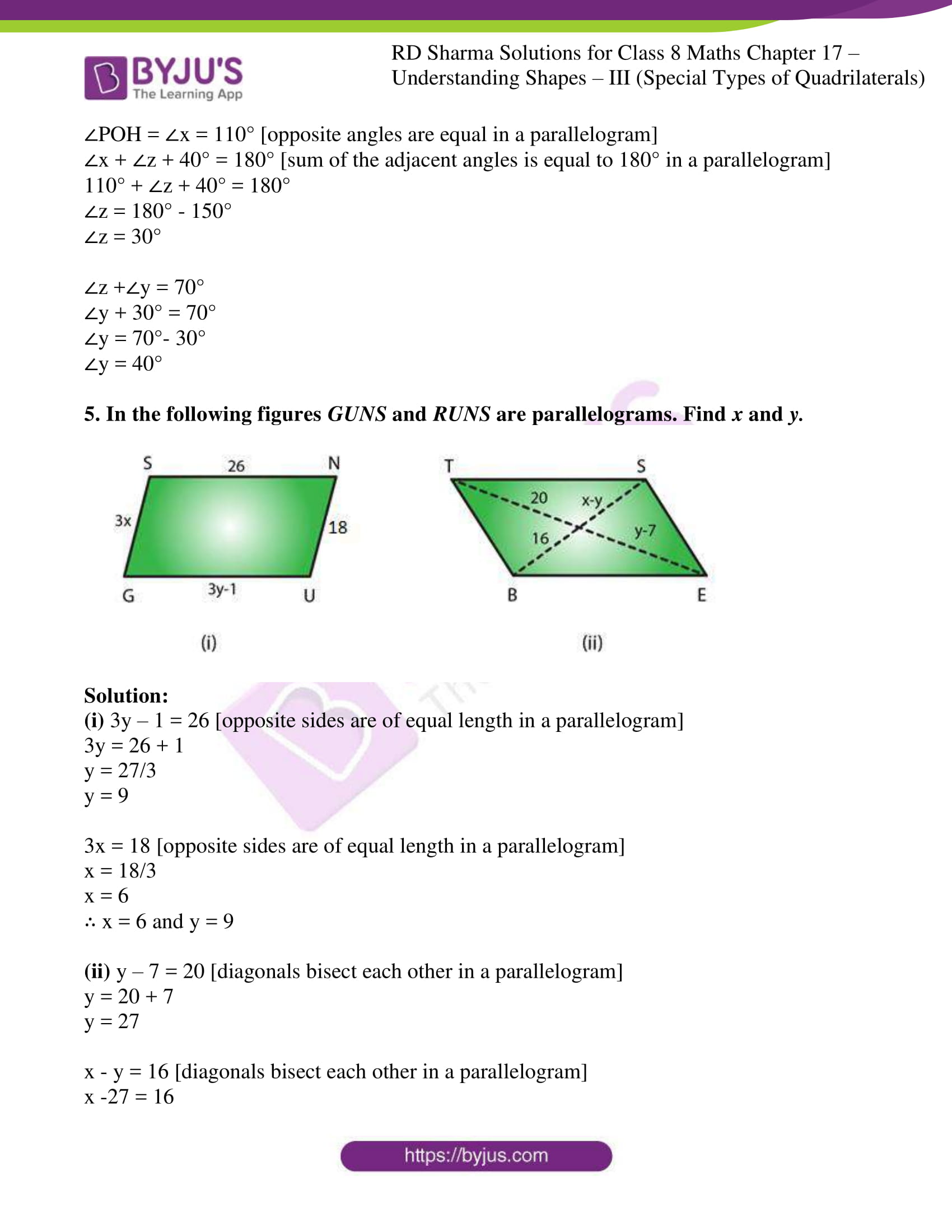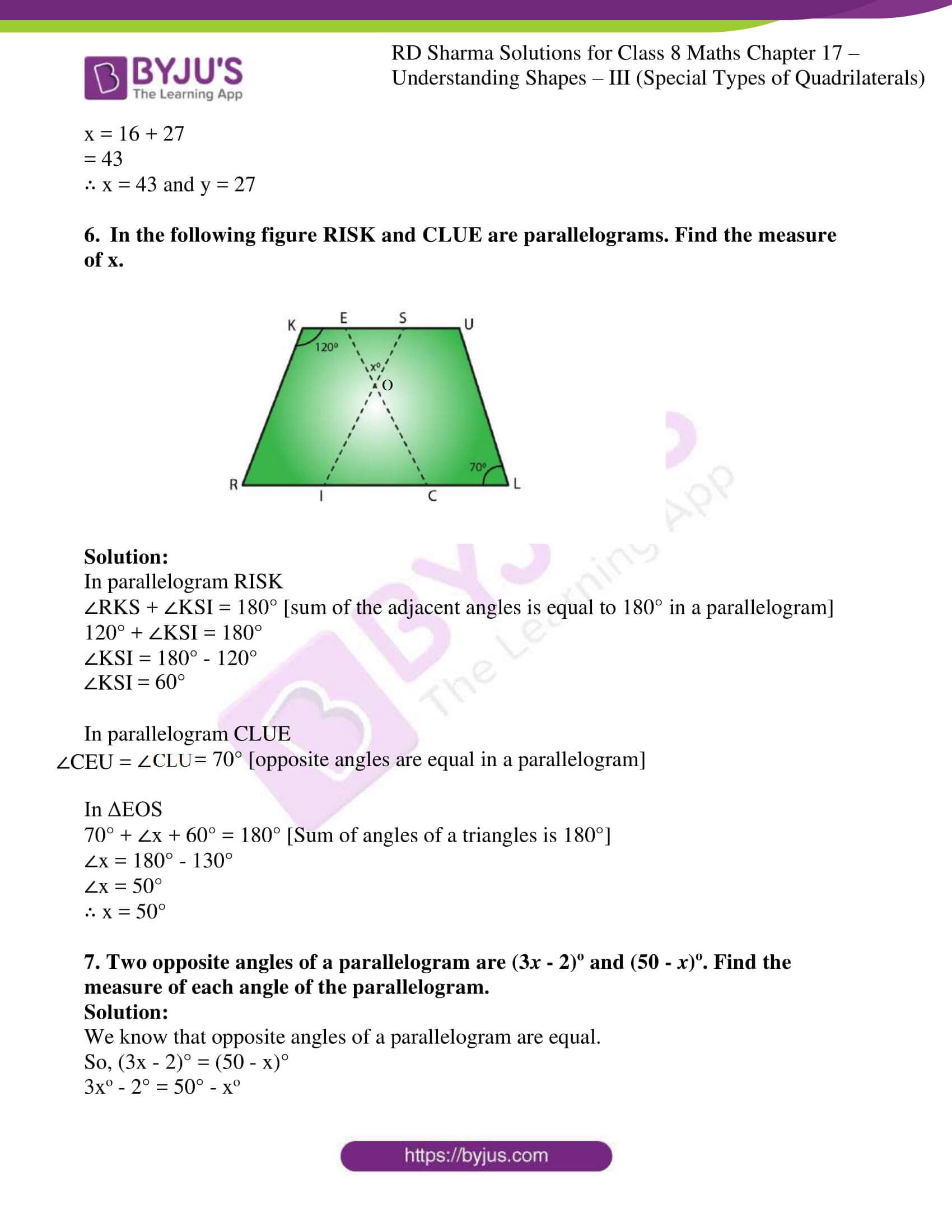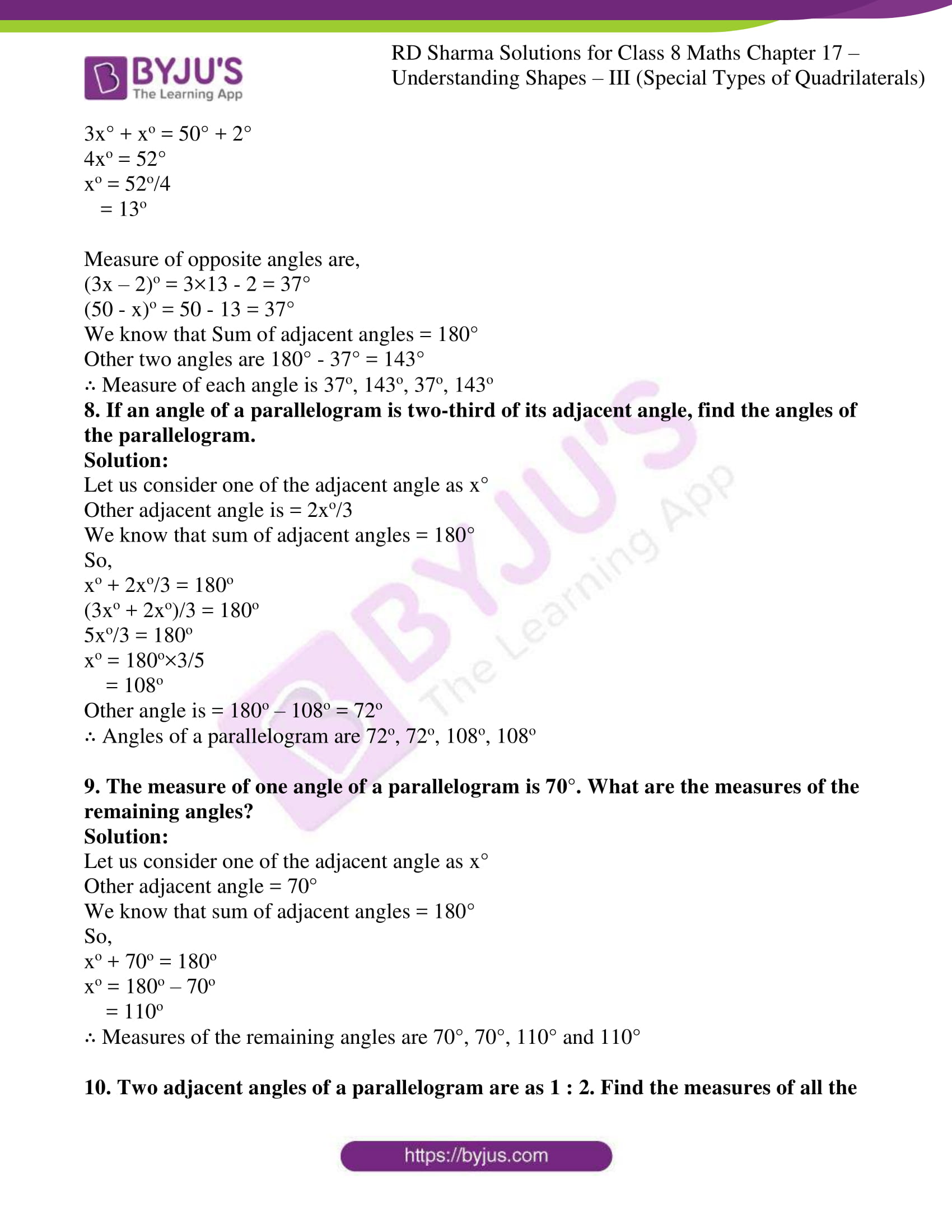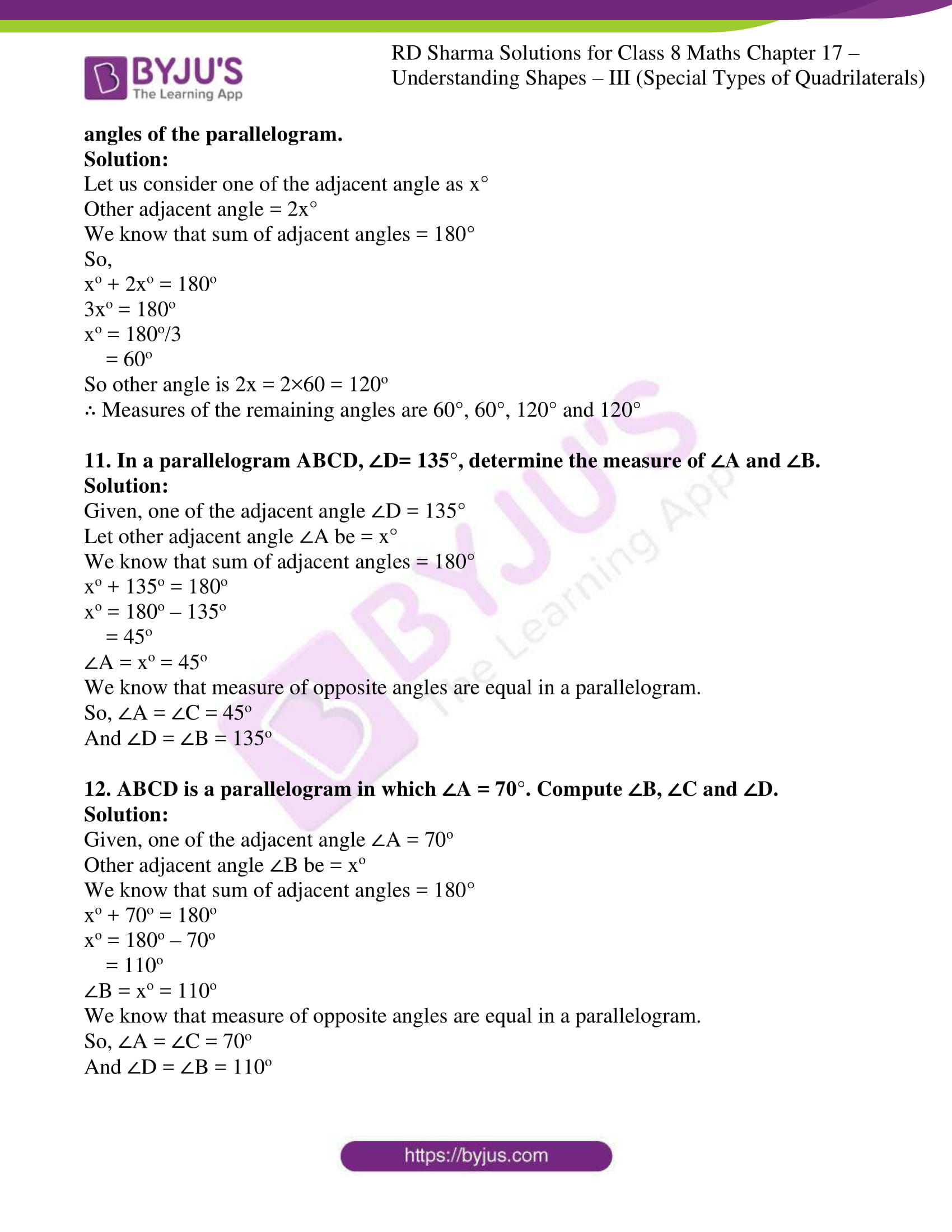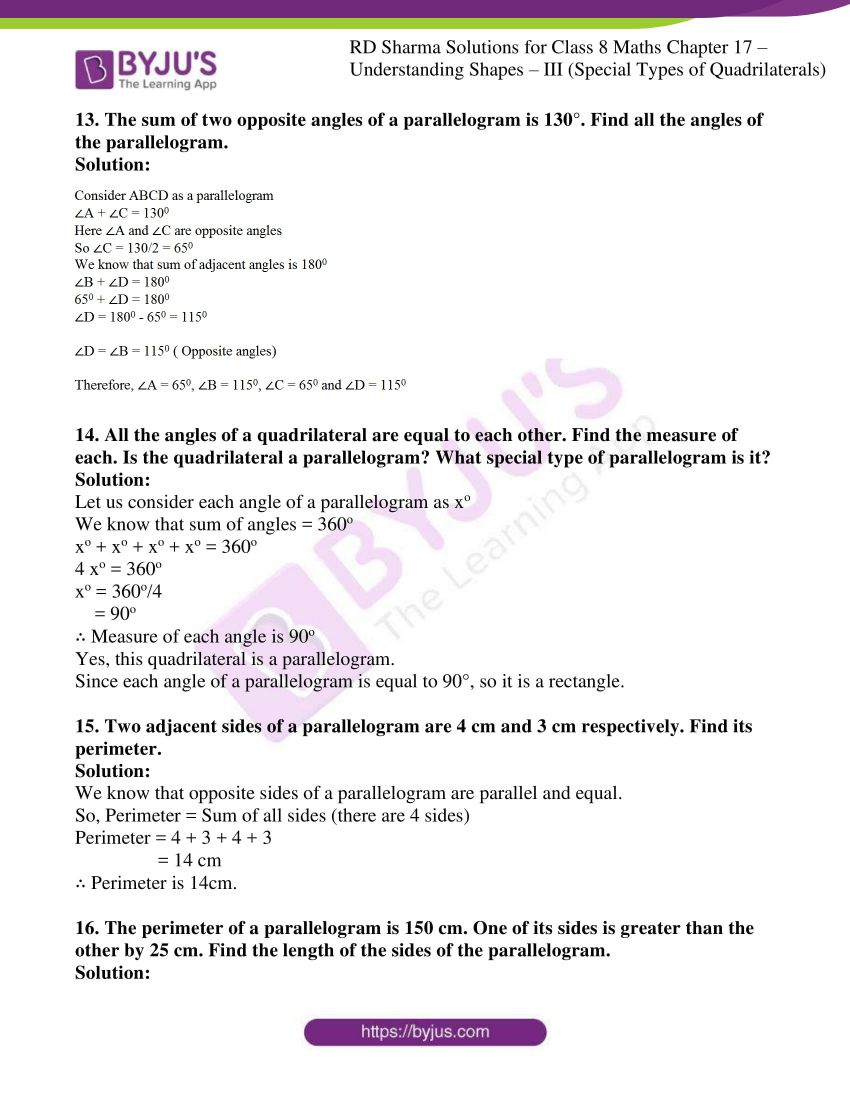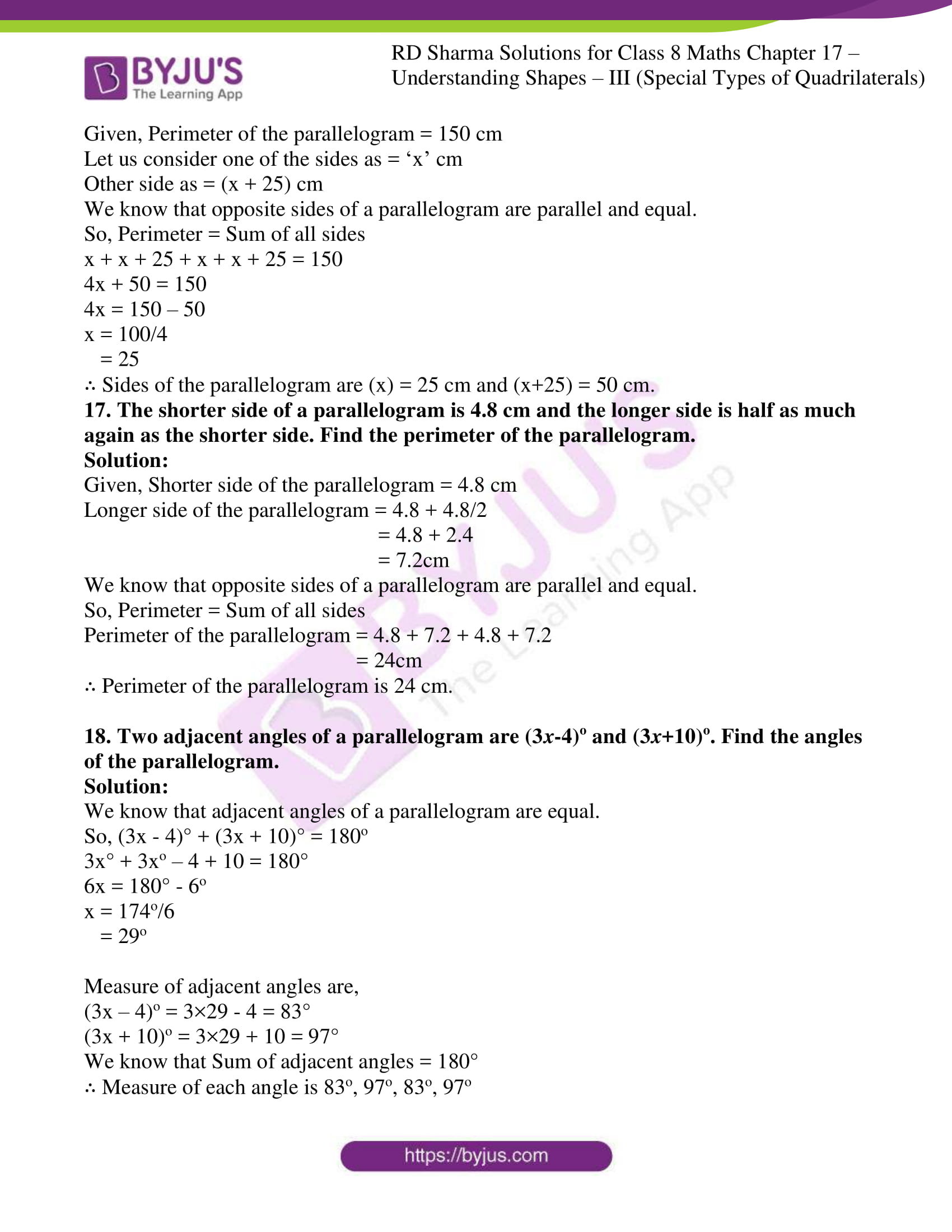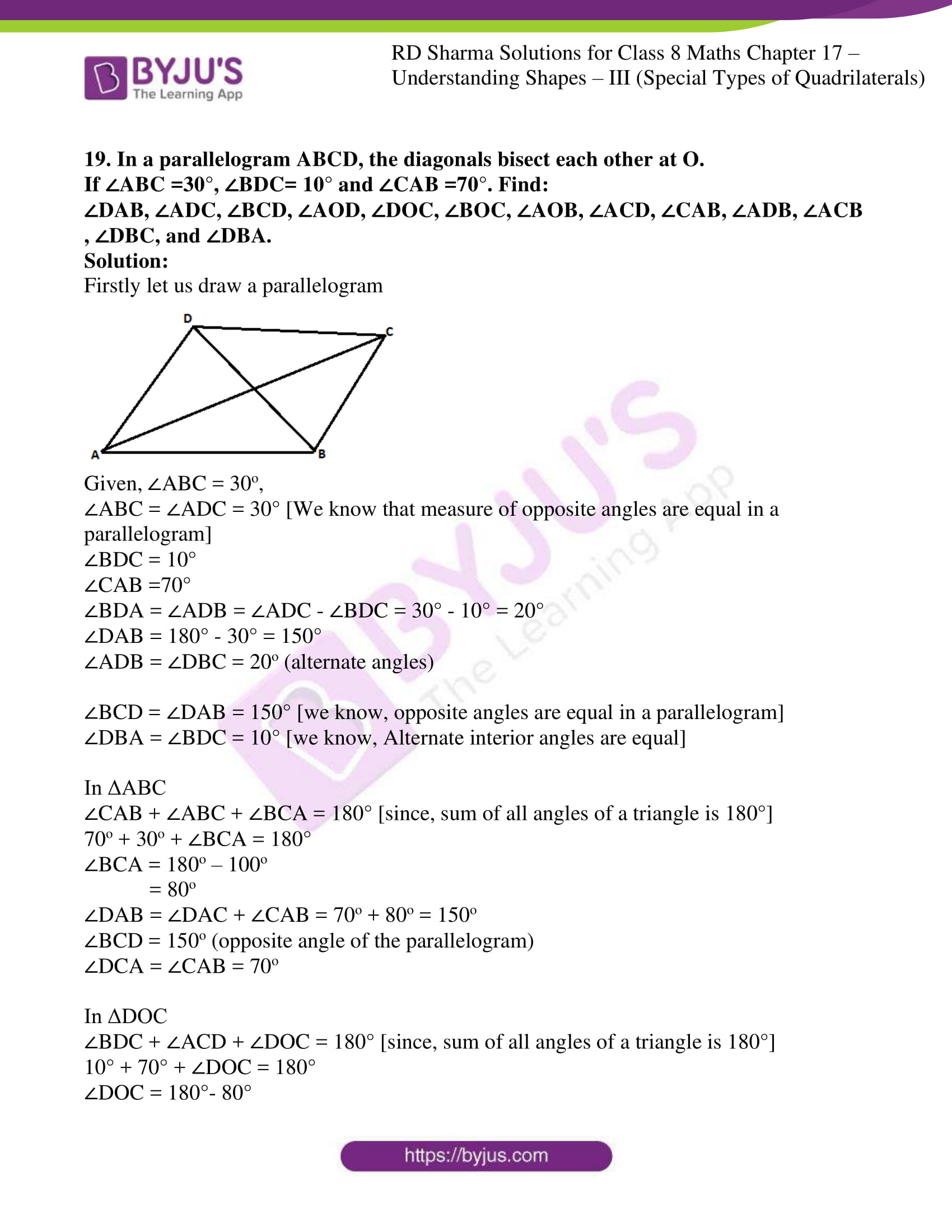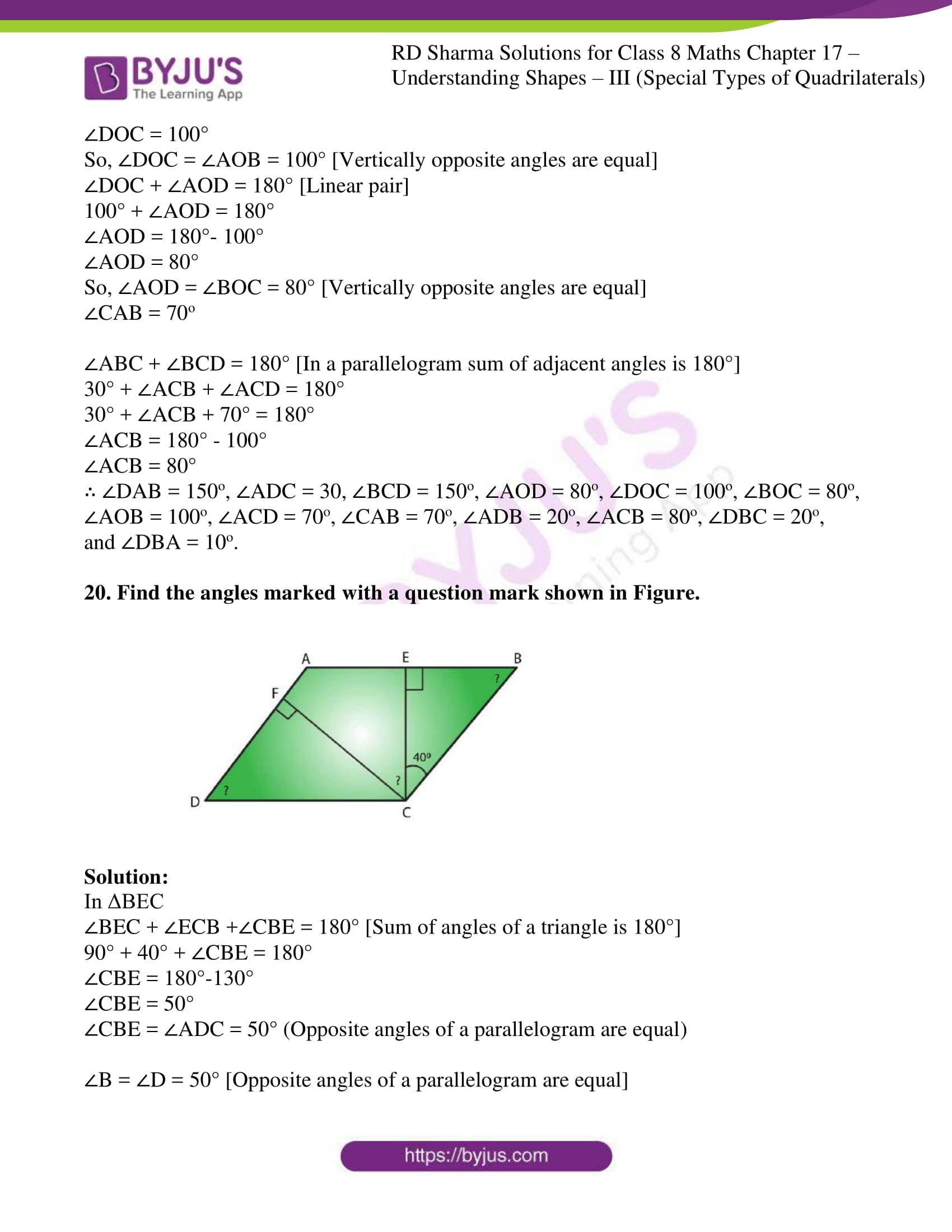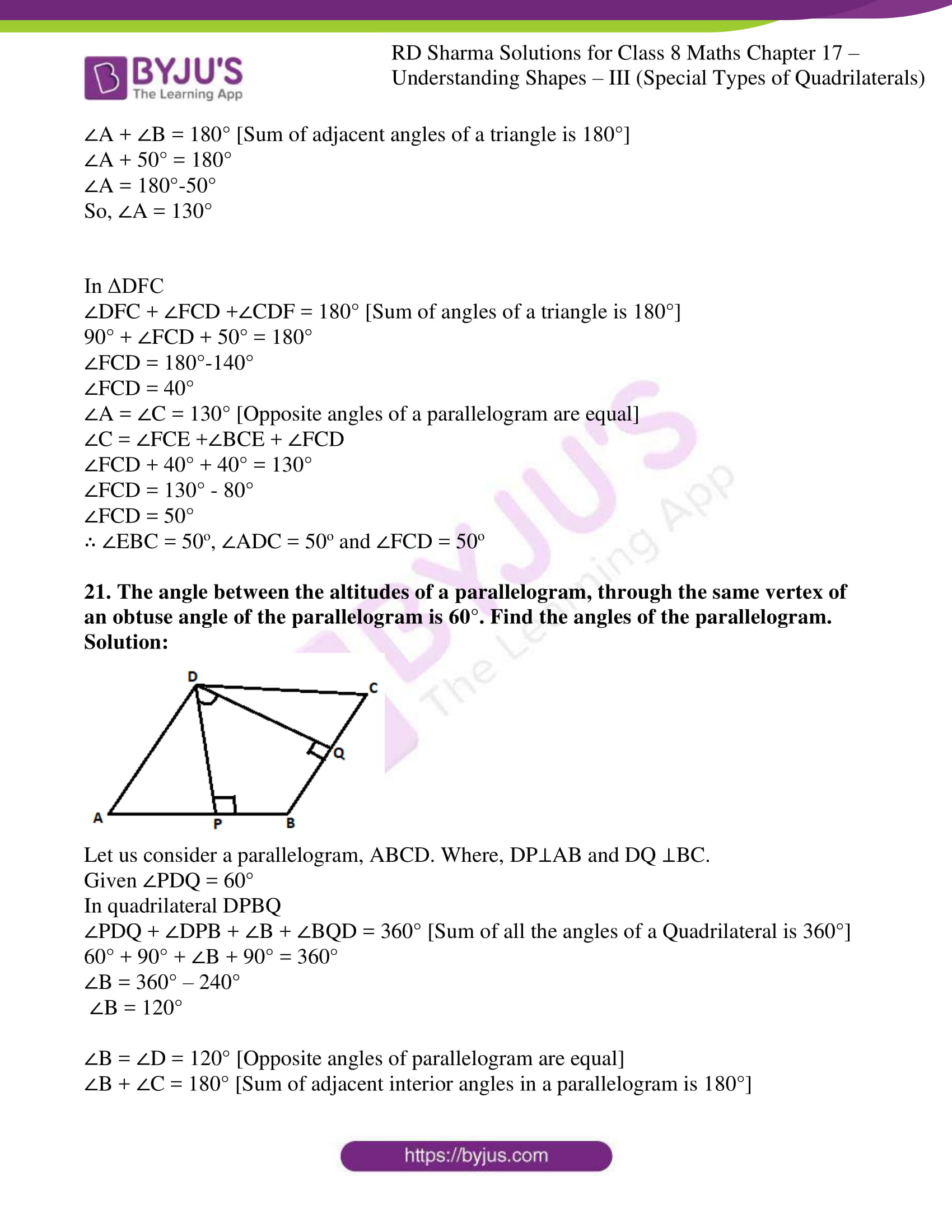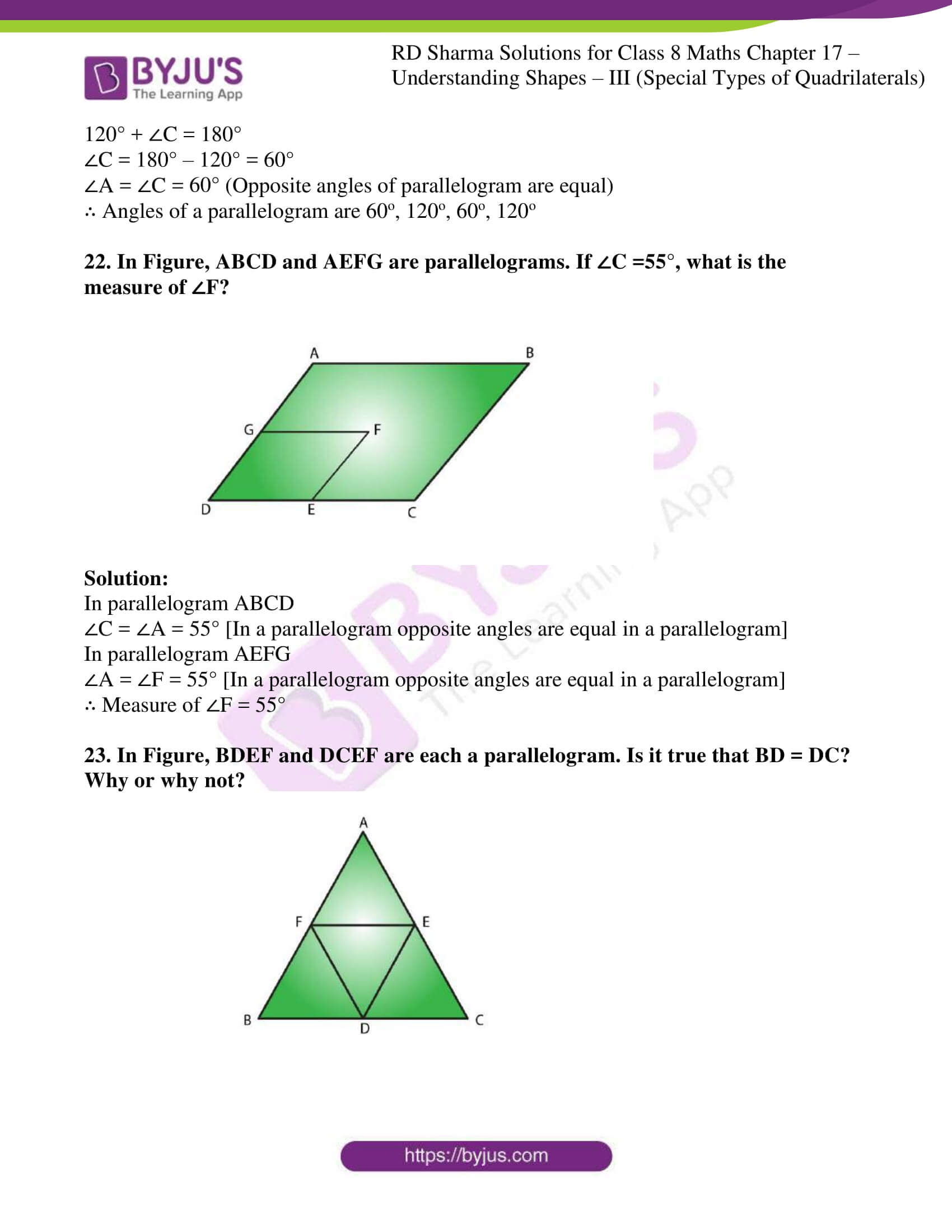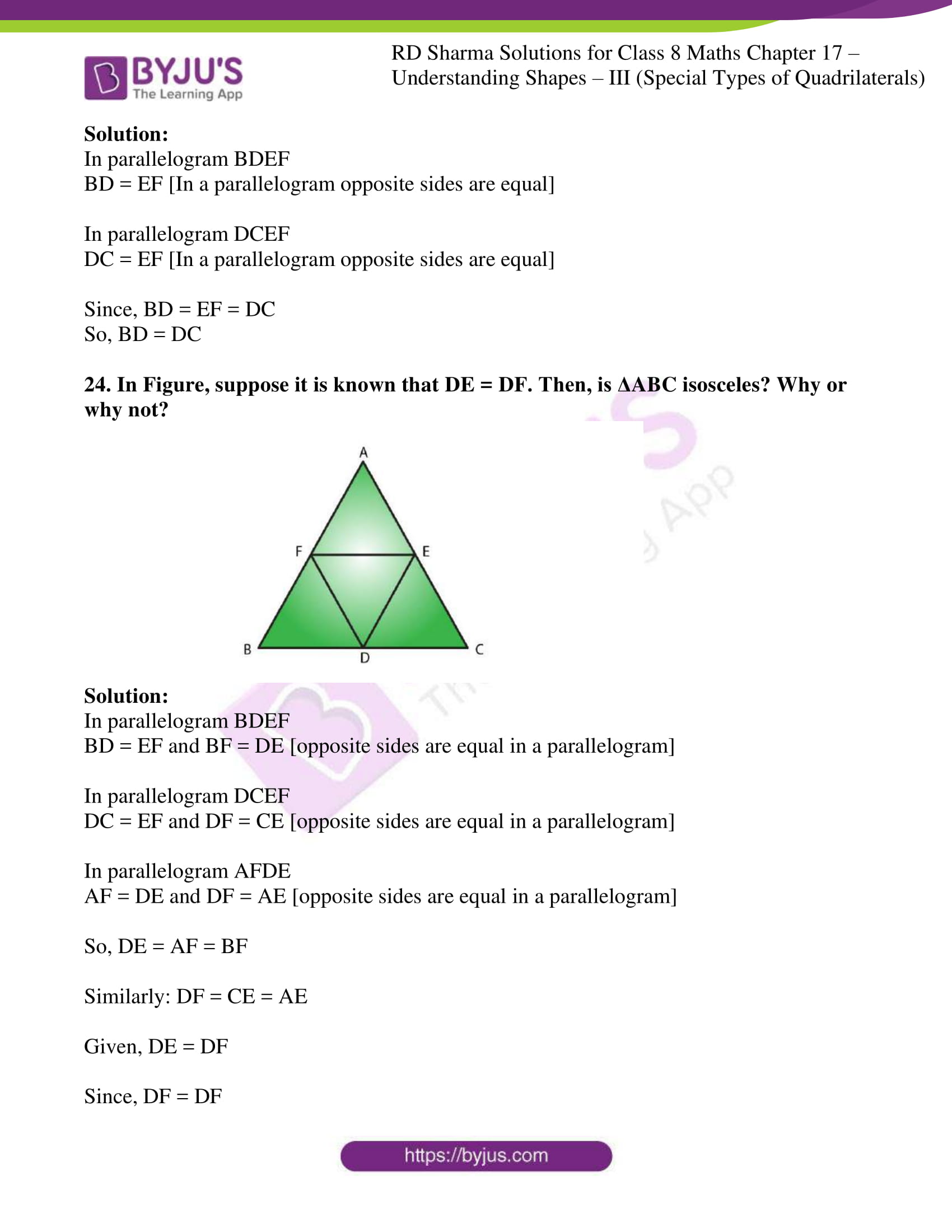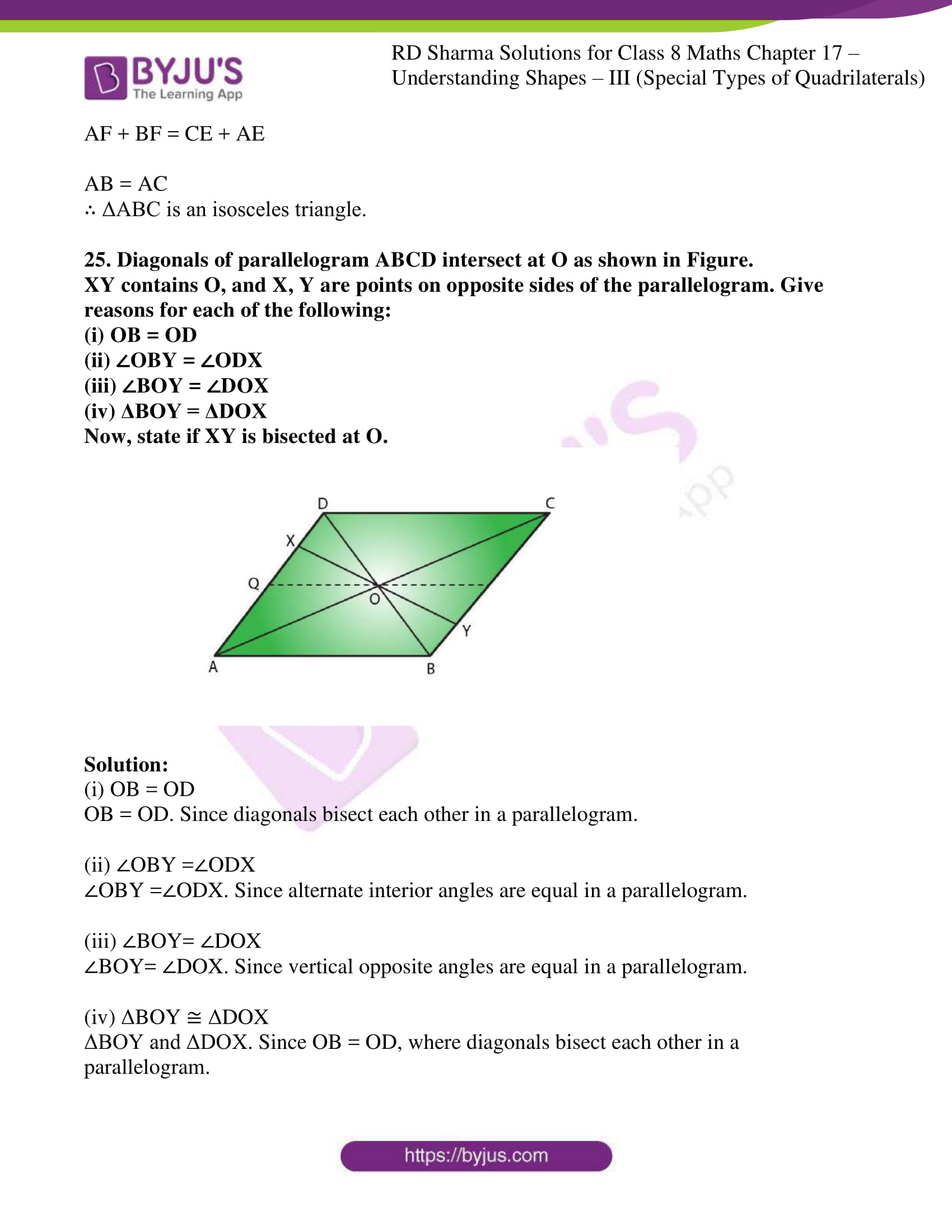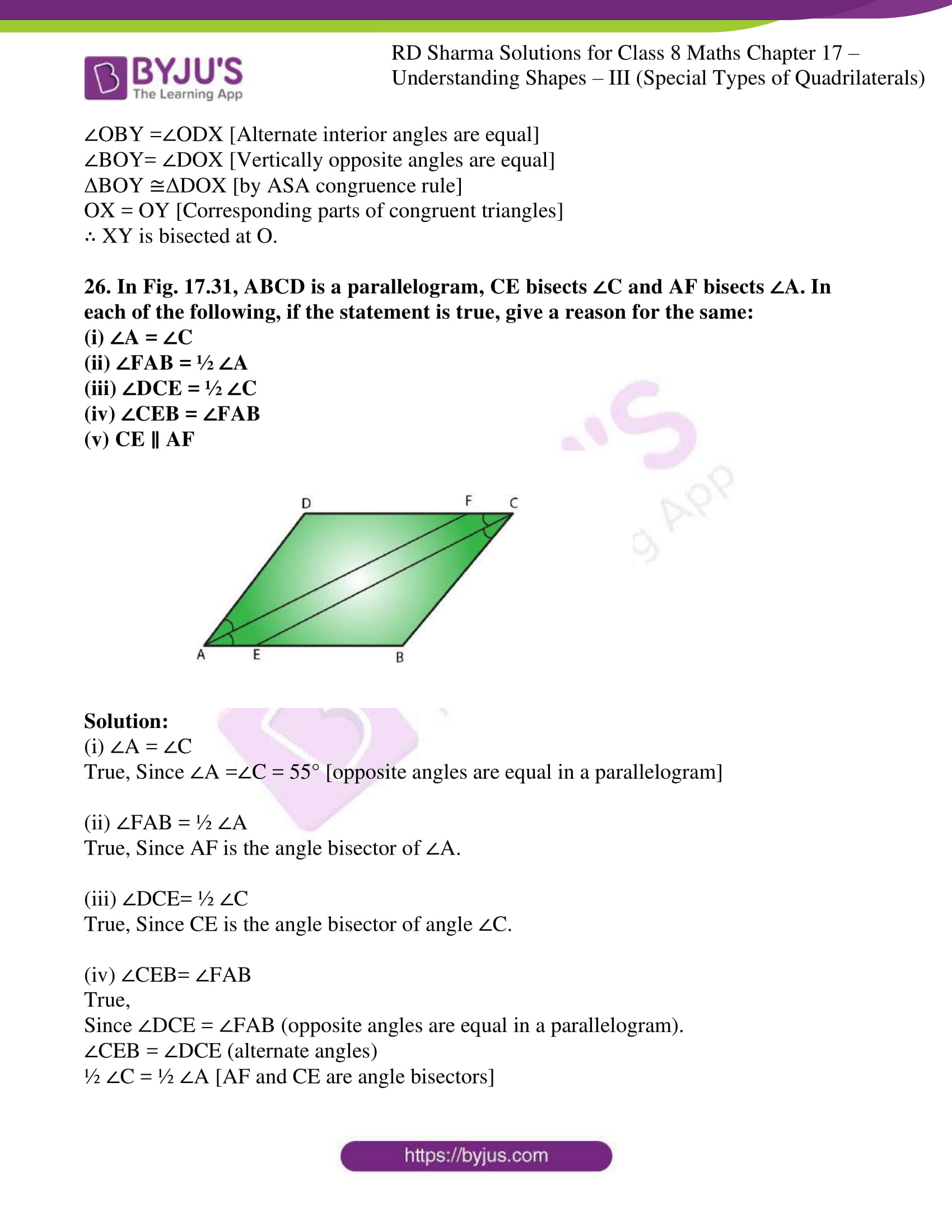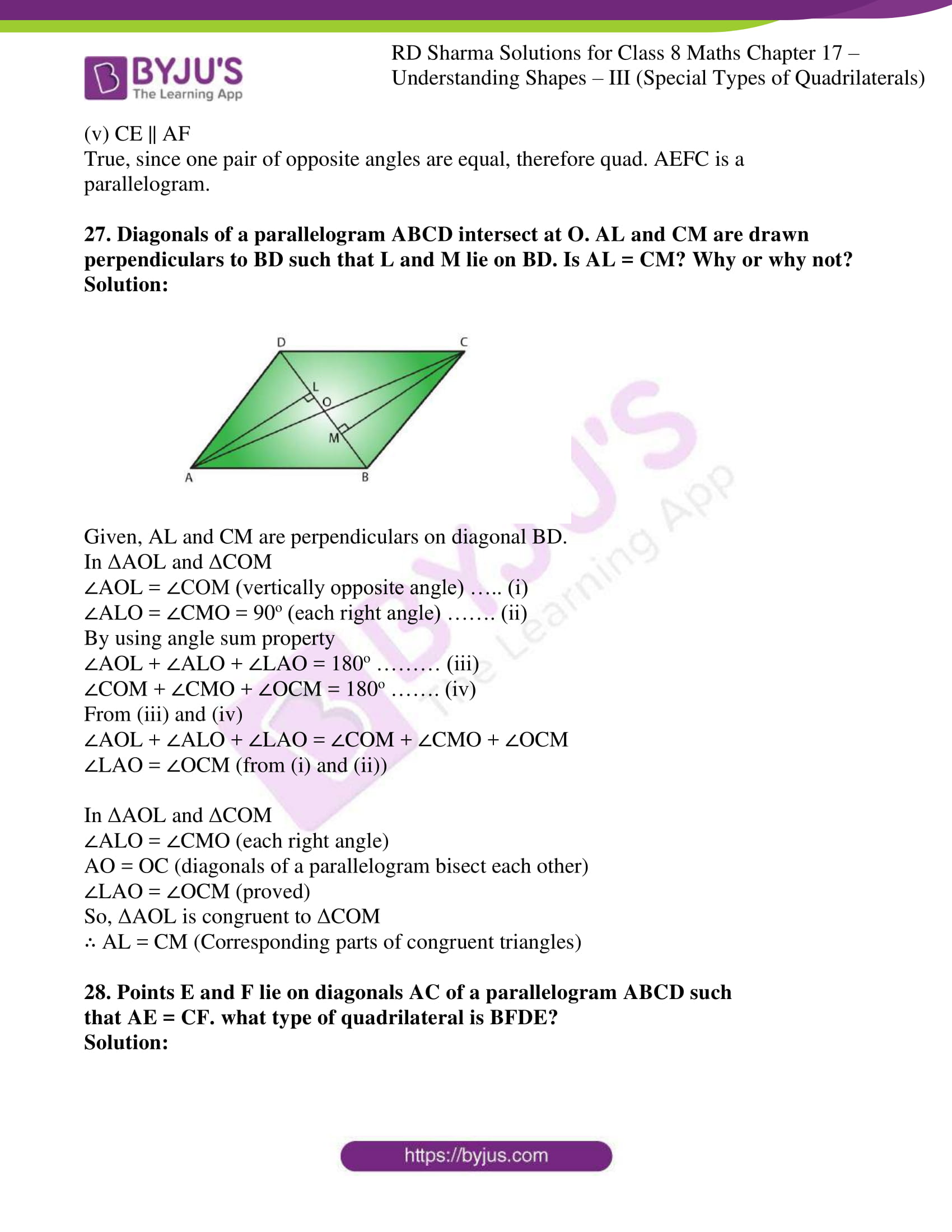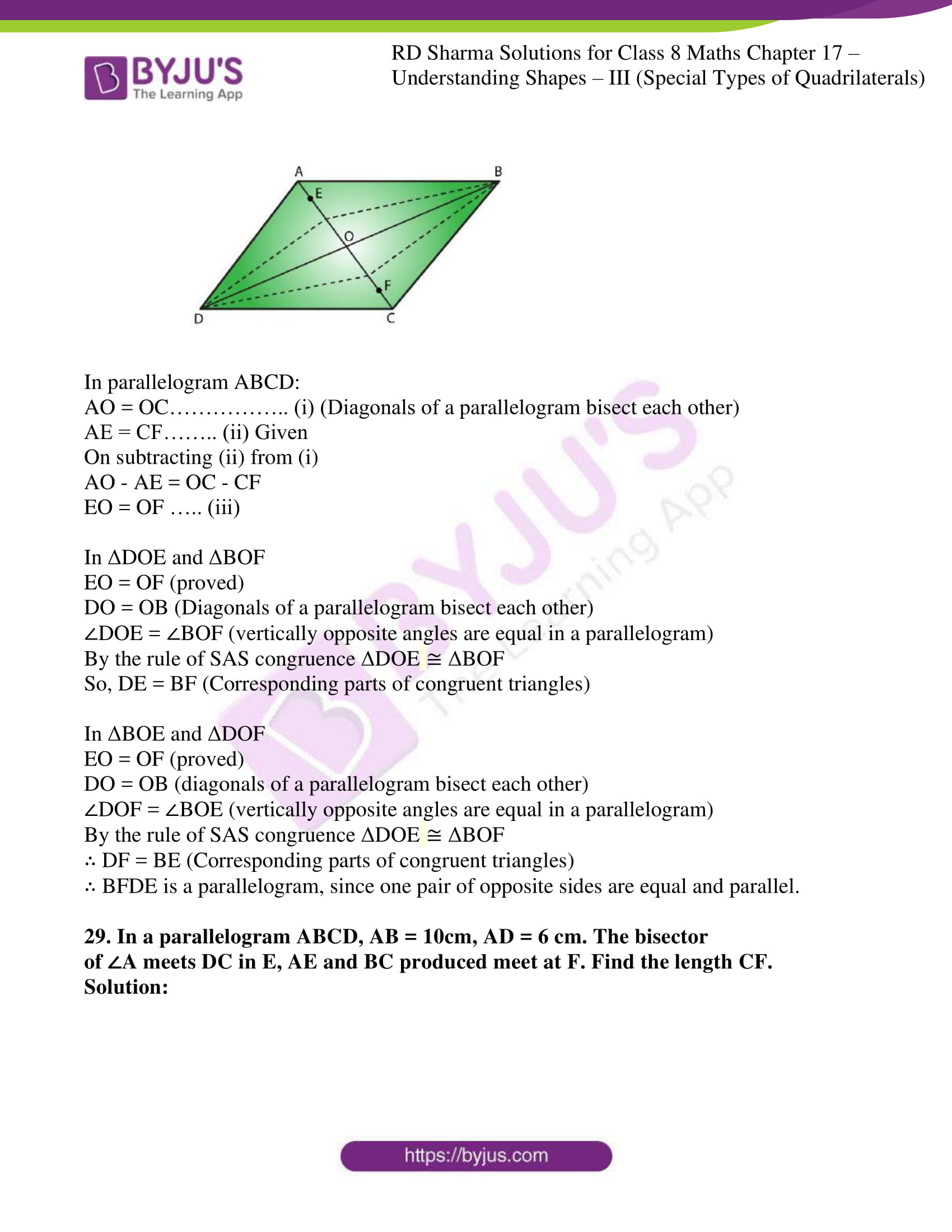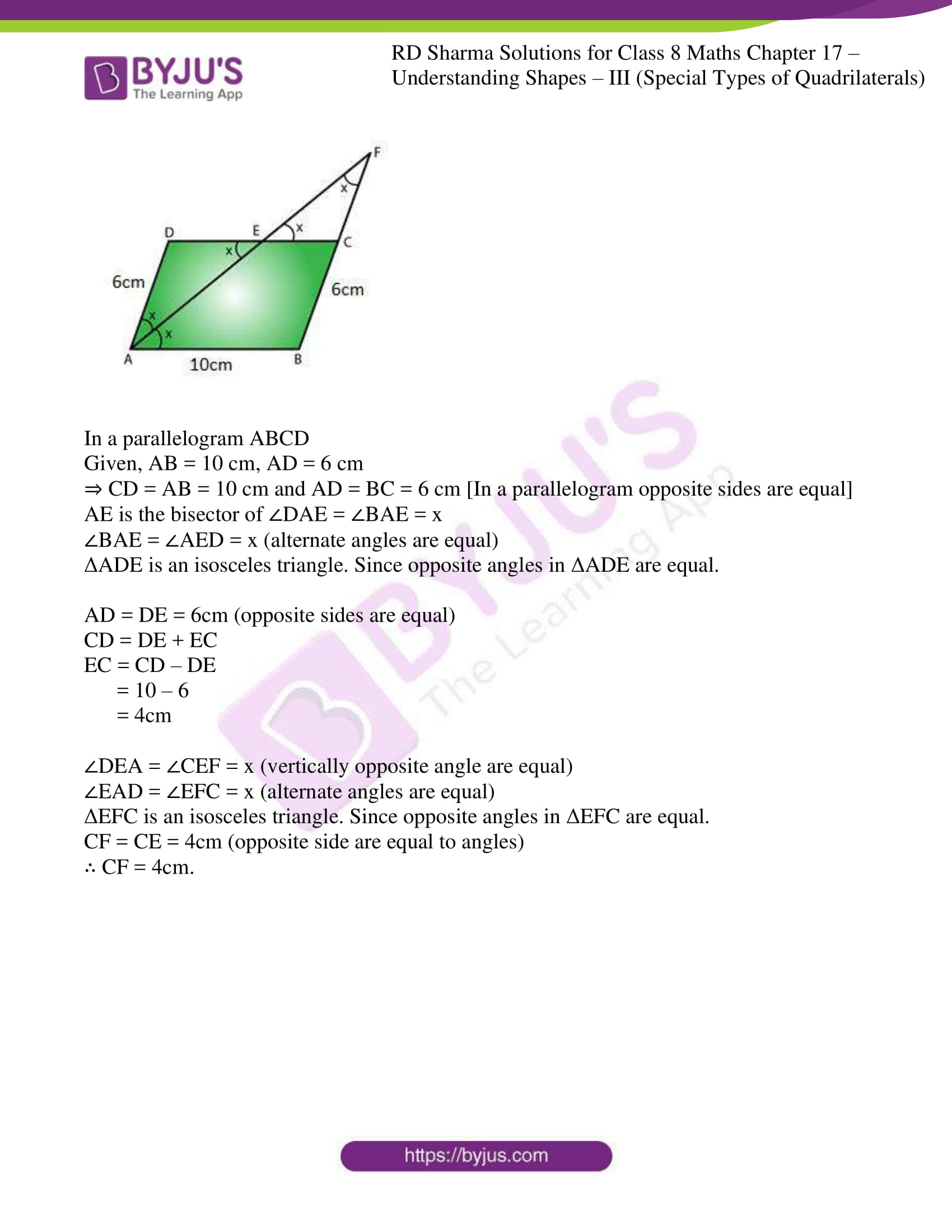### Access answers to Maths RD Sharma Solutions For Class 8 Exercise 17.1 Chapter 17 Understanding Shapes- III (Special Types of Quadrilaterals)

1. Given below is a parallelogram ABCD. Complete each statement along with the definition or property used.

(ii) ∠DCB =
(iii) OC =

(iv) ∠DAB + ∠CDA =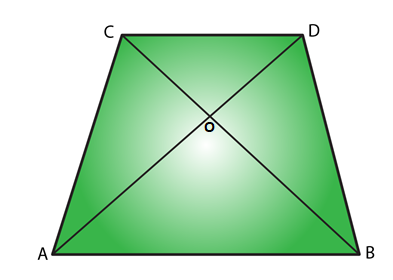Solution:

(i) AD = BC. Because, diagonals bisect each other in a parallelogram.

(ii) ∠DCB = ∠BAD. Because, alternate interior angles are equal.

(iii) OC = OA. Because, diagonals bisect each other in a parallelogram.

(iv) ∠DAB+ ∠CDA = 180°. Because sum of adjacent angles in a parallelogram is 180°.

2. The following figures are parallelograms. Find the degree values of the unknowns x, y, z.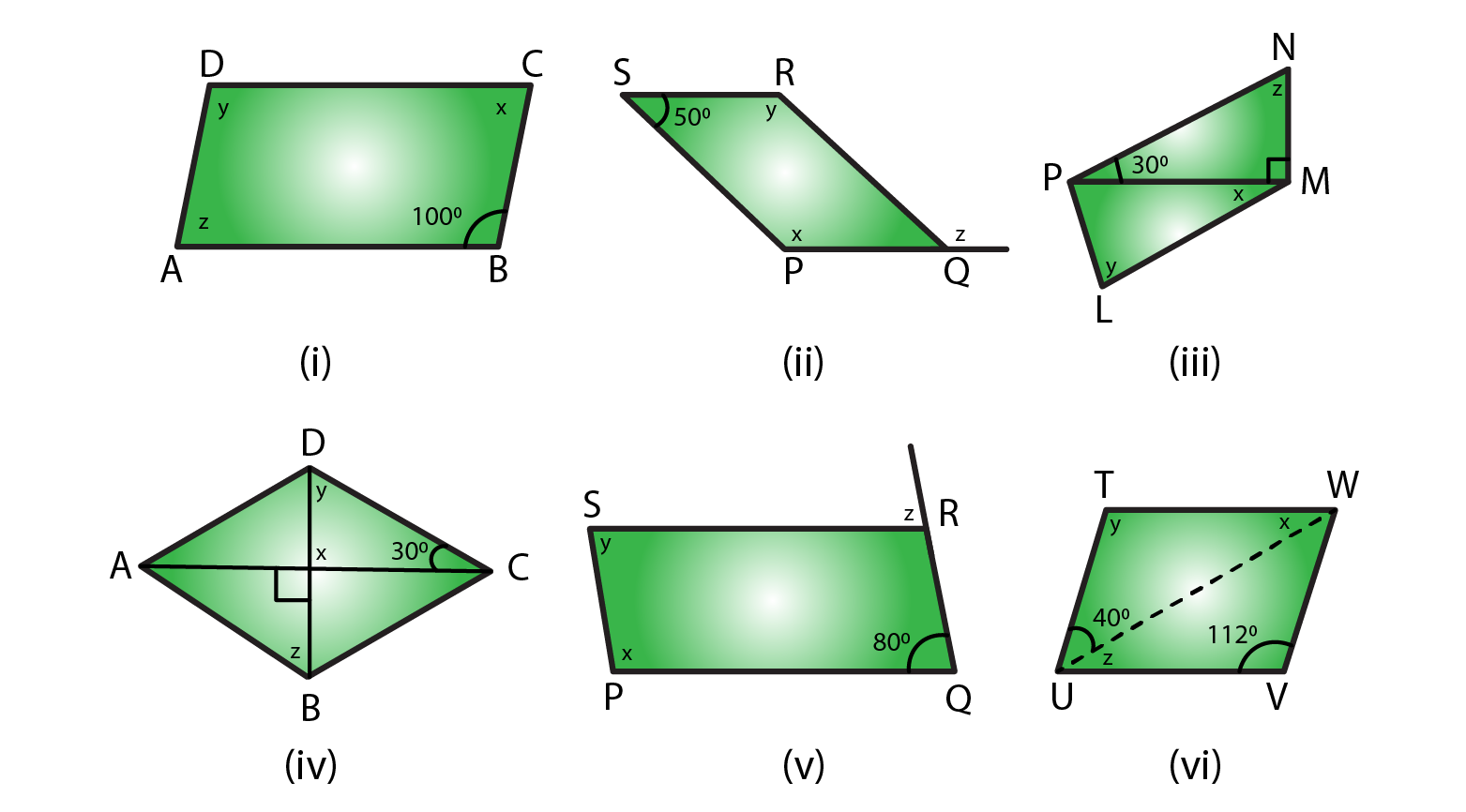Solution:

(i) ∠ABC = ∠y = 100o (opposite angles are equal in a parallelogram)

∠x + ∠y = 180o (sum of adjacent angles is = 180o in a parallelogram)

∠x + 100o = 180o

∠x = 180o – 100o

= 80o

∴ ∠x = 80o ∠y = 100o∠z = 80o (opposite angles are equal in a parallelogram)

(ii) ∠RSP + ∠y = 180o (sum of adjacent angles is = 180o in a parallelogram)

∠y + 50o = 180o

∠y = 180o – 50o

= 130o

∴ ∠x = ∠y = 130o (opposite angles are equal in a parallelogram)

∠RSP = ∠RQP = 50o (opposite angles are equal in a parallelogram)

∠RQP + ∠z = 180o (linear pair)

50o + ∠z = 180o

∠z = 180o – 50o

= 130o

∴ ∠x = 130o ∠y = 130o ∠z = 130o

(iii) In ΔPMN

∠NPM + ∠NMP + ∠MNP = 180° [Sum of all the angles of a triangle is 180°]

30° + 90° + ∠z = 180°

∠z = 180°-120°

= 60°

∠y = ∠z = 60° [opposite angles are equal in a parallelogram]

∠z = 180°-120° [sum of the adjacent angles is equal to 180° in a parallelogram]

∠z = 60°

∠z + ∠LMN = 180° [sum of the adjacent angles is equal to 180° in a parallelogram]

60° + 90°+ ∠x = 180°

∠x = 180°-150°

∠x = 30°

∴ ∠x = 30o ∠y = 60o ∠z = 60o

(iv) ∠x = 90° [vertically opposite angles are equal]

In ΔDOC

∠x + ∠y + 30° = 180° [Sum of all the angles of a triangle is 180°]

90° + 30° + ∠y = 180°

∠y = 180°-120°

∠y = 60°

∠y = ∠z = 60° [alternate interior angles are equal]

∴ ∠x = 90o ∠y = 60o ∠z = 60o

(v) ∠x + ∠POR = 180° [sum of the adjacent angles is equal to 180° in a parallelogram]

∠x + 80° = 180°

∠x = 180°-80°

∠x = 100°

∠y = 80° [opposite angles are equal in a parallelogram]

∠SRQ =∠x = 100°

∠SRQ + ∠z = 180° [Linear pair]

100° + ∠z = 180°

∠z = 180°-100°

∠z = 80°

∴ ∠x = 100o ∠y = 80o ∠z = 80o

(vi) ∠y = 112° [In a parallelogram opposite angles are equal]

∠y + ∠VUT = 180° [In a parallelogram sum of the adjacent angles is equal to 180°]

∠z + 40° + 112° = 180°

∠z = 180°-152°

∠z = 28°

∠z =∠x = 28° [alternate interior angles are equal]

∴ ∠x = 28o ∠y = 112o ∠z = 28o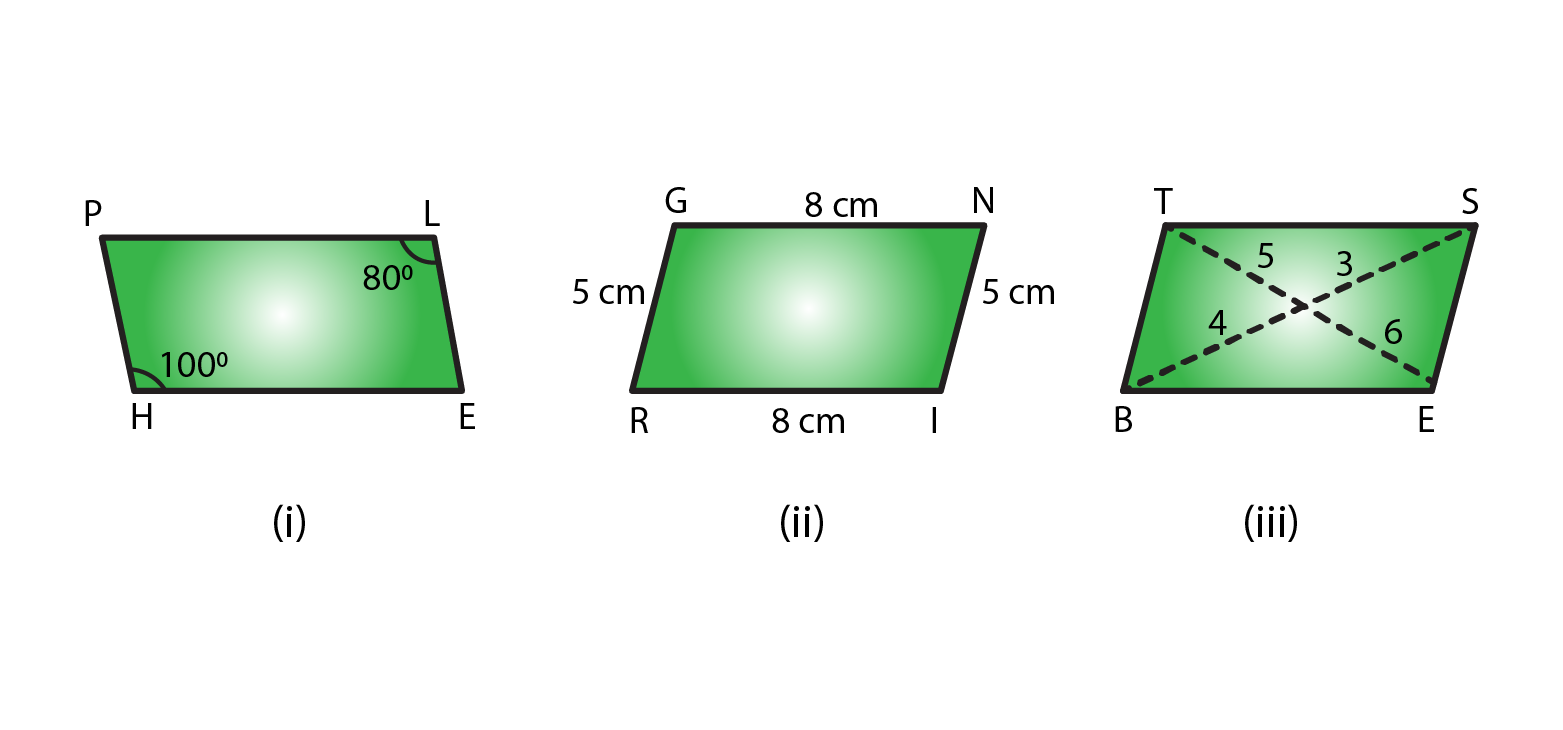Solution:

(i) No, opposite angles are equal in a parallelogram.

(ii) Yes, opposite sides are equal and parallel in a parallelogram.

(iii) No, diagonals bisect each other in a parallelogram.

4. In the adjacent figure HOPE is a parallelogram. Find the angle measures x, y and z. State the geometrical truths you use to find them.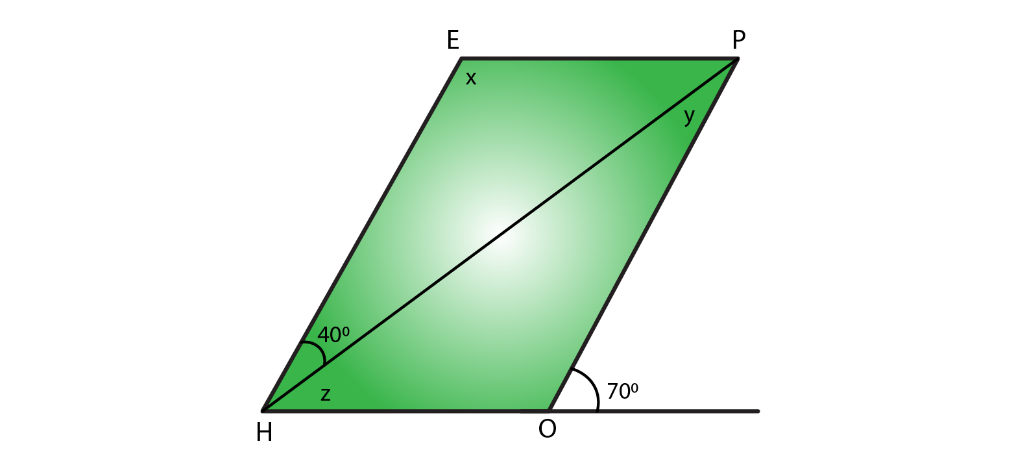Solution:

We know that

∠POH + 70° = 180° [Linear pair]

∠POH = 180°-70°

∠POH = 110°

∠POH = ∠x = 110° [opposite angles are equal in a parallelogram]

∠x + ∠z + 40° = 180° [sum of the adjacent angles is equal to 180° in a parallelogram]

110° + ∠z + 40° = 180°

∠z = 180° – 150°

∠z = 30°

∠z +∠y = 70°

∠y + 30° = 70°

∠y = 70°- 30°

∠y = 40°

5. In the following figures GUNS and RUNS are parallelograms. Find x and y.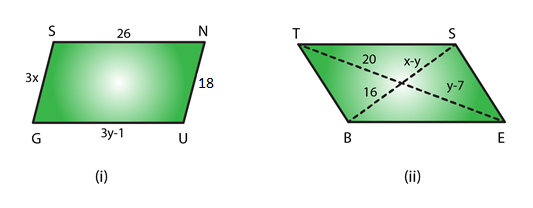Solution:

(i) 3y – 1 = 26 [opposite sides are of equal length in a parallelogram]

3y = 26 + 1

y = 27/3

y = 9

3x = 18 [opposite sides are of equal length in a parallelogram]

x = 18/3

x = 6

∴ x = 6 and y = 9

(ii) y – 7 = 20 [diagonals bisect each other in a parallelogram]

y = 20 + 7

y = 27

x – y = 16 [diagonals bisect each other in a parallelogram]

x -27 = 16

x = 16 + 27

= 43

∴ x = 43 and y = 27

6. In the following figure RISK and CLUE are parallelograms. Find the measure of x.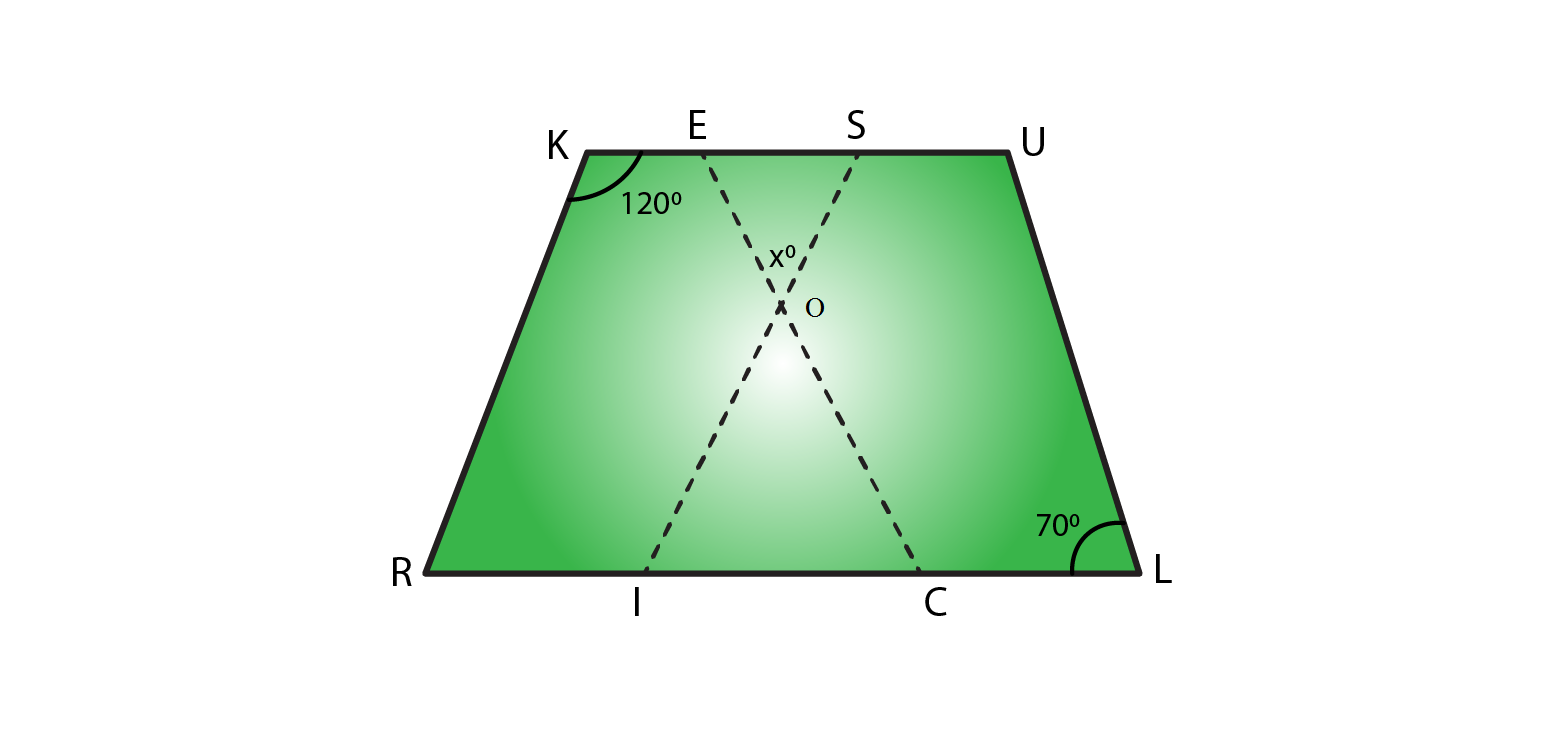Solution:

In parallelogram RISK

∠RKS + ∠KSI = 180° [sum of the adjacent angles is equal to 180° in a parallelogram]

120° + ∠KSI = 180°

∠KSI = 180° – 120°

∠KSI = 60°

In parallelogram CLUE

∠CEU = ∠CLU = 70° [opposite angles are equal in a parallelogram]

In ΔEOS

70° + ∠x + 60° = 180° [Sum of angles of a triangles is 180°]

∠x = 180° – 130°

∠x = 50°

∴ x = 50°

7. Two opposite angles of a parallelogram are (3– 2)o and (50 – x)o. Find the measure of each angle of the parallelogram.

Solution:

We know that opposite angles of a parallelogram are equal.

So, (3x – 2)° = (50 – x)°

3xo – 2° = 50° – xo

3x° + xo = 50° + 2°

4xo = 52°

xo = 52o/4

= 13o

Measure of opposite angles are,

(3x – 2)o = 3×13 – 2 = 37°

(50 – x)o = 50 – 13 = 37°

We know that Sum of adjacent angles = 180°

Other two angles are 180° – 37° = 143°

∴ Measure of each angle is 37o, 143o, 37o, 143o

8. If an angle of a parallelogram is two-third of its adjacent angle, find the angles of the parallelogram.

Solution:

Let us consider one of the adjacent angle as x°

Other adjacent angle is = 2xo/3

We know that sum of adjacent angles = 180°

So,

xo + 2xo/3 = 180o

(3xo + 2xo)/3 = 180o

5xo/3 = 180o

xo = 180o×3/5

= 108o

Other angle is = 180o – 108o = 72o

∴ Angles of a parallelogram are 72o, 72o, 108o, 108o

9. The measure of one angle of a parallelogram is 70°. What are the measures of the remaining angles?

Solution:

Let us consider one of the adjacent angle as x°

We know that sum of adjacent angles = 180°

So,

xo + 70o = 180o

xo = 180o – 70o

= 110o

∴ Measures of the remaining angles are 70°, 70°, 110° and 110°

10. Two adjacent angles of a parallelogram are as 1 : 2. Find the measures of all the angles of the parallelogram.

Solution:

Let us consider one of the adjacent angle as x°

We know that sum of adjacent angles = 180°

So,

xo + 2xo = 180o

3xo = 180o

xo = 180o/3

= 60o

So other angle is 2x = 2×60 = 120o

∴ Measures of the remaining angles are 60°, 60°, 120° and 120°

11. In a parallelogram ABCD, ∠D= 135°, determine the measure of ∠A and ∠B.

Solution:

Given, one of the adjacent angle ∠D = 135°

Let other adjacent angle ∠A be = x°

We know that sum of adjacent angles = 180°

xo + 135o = 180o

xo = 180o – 135o

= 45o

∠A = xo = 45o

We know that measure of opposite angles are equal in a parallelogram.

So, ∠A = ∠C = 45o

And ∠D = ∠B = 135o

12. ABCD is a parallelogram in which ∠A = 70°. Compute ∠B, ∠C and ∠D.

Solution:

Given, one of the adjacent angle ∠A = 70o

Other adjacent angle ∠B be = xo

We know that sum of adjacent angles = 180°

xo + 70o = 180o

xo = 180o – 70o

= 110o

∠B = xo = 110o

We know that measure of opposite angles are equal in a parallelogram.

So, ∠A = ∠C = 70o

And ∠D = ∠B = 110o

13. The sum of two opposite angles of a parallelogram is 130°. Find all the angles of the parallelogram.

Solution:

Consider ABCD as a parallelogram

∠A + ∠C = 1300

Here ∠A and ∠C are opposite angles

So ∠C = 130/2 = 650

We know that sum of adjacent angles is 1800

∠B + ∠D = 1800

650 + ∠D = 1800

∠D = 1800 – 650 = 1150

∠D = ∠B = 1150 ( Opposite angles)

Therefore, ∠A = 650, ∠B = 1150, ∠C = 650 and ∠D = 1150.

14. All the angles of a quadrilateral are equal to each other. Find the measure of each. Is the quadrilateral a parallelogram? What special type of parallelogram is it?

Solution:

Let us consider each angle of a parallelogram as xo

We know that sum of angles = 360o

xo + xo + xo + xo = 360o

4 xo = 360o

xo = 360o/4

= 90o

∴ Measure of each angle is 90o

Yes, this quadrilateral is a parallelogram.

Since each angle of a parallelogram is equal to 90°, so it is a rectangle.

15. Two adjacent sides of a parallelogram are 4 cm and 3 cm respectively. Find its perimeter.

Solution:

We know that opposite sides of a parallelogram are parallel and equal.

So, Perimeter = Sum of all sides (there are 4 sides)

Perimeter = 4 + 3 + 4 + 3

= 14 cm

∴ Perimeter is 14cm.

16. The perimeter of a parallelogram is 150 cm. One of its sides is greater than the other by 25 cm. Find the length of the sides of the parallelogram.

Solution:

Given, Perimeter of the parallelogram = 150 cm

Let us consider one of the sides as = ‘x’ cm

Other side as = (x + 25) cm

We know that opposite sides of a parallelogram are parallel and equal.

So, Perimeter = Sum of all sides

x + x + 25 + x + x + 25 = 150

4x + 50 = 150

4x = 150 – 50

x = 100/4

= 25

∴ Sides of the parallelogram are (x) = 25 cm and (x+25) = 50 cm.

17. The shorter side of a parallelogram is 4.8 cm and the longer side is half as much again as the shorter side. Find the perimeter of the parallelogram.

Solution:

Given, Shorter side of the parallelogram = 4.8 cm

Longer side of the parallelogram = 4.8 + 4.8/2

= 4.8 + 2.4

= 7.2cm

We know that opposite sides of a parallelogram are parallel and equal.

So, Perimeter = Sum of all sides

Perimeter of the parallelogram = 4.8 + 7.2 + 4.8 + 7.2

= 24cm

∴ Perimeter of the parallelogram is 24 cm.

18. Two adjacent angles of a parallelogram are (3x-4)o and (3x+10)o. Find the angles of the parallelogram.

Solution:

We know that adjacent angles of a parallelogram are equal.

So, (3x – 4)° + (3x + 10)° = 180o

3x° + 3xo – 4 + 10 = 180°

6x = 180° – 6o

x = 174o/6

= 29o

(3x – 4)o = 3×29 – 4 = 83°

(3x + 10)o = 3×29 + 10 = 97°

We know that Sum of adjacent angles = 180°

∴ Measure of each angle is 83o, 97o, 83o, 97o

19. In a parallelogram ABCD, the diagonals bisect each other at O.  If ∠ABC =30°, ∠BDC= 10° and ∠CAB =70°. Find:
∠DAB, ∠ADC, ∠BCD, ∠AOD, ∠DOC, ∠BOC, ∠AOB, ∠ACD, ∠CAB, ∠ADB, ∠ACB, ∠DBC, and ∠DBA.

Solution:

Firstly let us draw a parallelogram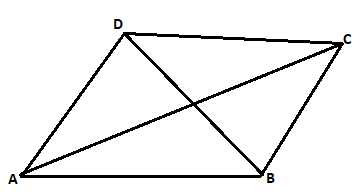Given, ∠ABC = 30o,

∠ABC = ∠ADC = 30° [We know that measure of opposite angles are equal in a parallelogram]

∠BDC = 10°

∠CAB =70°

∠BDA = ∠ADB = ∠ADC – ∠BDC = 30° – 10° = 20°

∠DAB = 180° – 30° = 150°

∠ADB = ∠DBC = 20o (alternate angles)

∠BCD = ∠DAB = 150° [we know, opposite angles are equal in a parallelogram]

∠DBA = ∠BDC = 10° [we know, Alternate interior angles are equal]

In ΔABC

∠CAB + ∠ABC + ∠BCA = 180° [since, sum of all angles of a triangle is 180°]

70o + 30o + ∠BCA = 180°

∠BCA = 180o – 100o

= 80o

∠DAB = ∠DAC + ∠CAB = 70o + 80o = 150o

∠BCD = 150o (opposite angle of the parallelogram)

∠DCA = ∠CAB = 70o

In ΔDOC

∠BDC + ∠ACD + ∠DOC = 180° [since, sum of all angles of a triangle is 180°]

10° + 70° + ∠DOC = 180°

∠DOC = 180°- 80°

∠DOC = 100°

So, ∠DOC = ∠AOB = 100° [Vertically opposite angles are equal]

∠DOC + ∠AOD = 180° [Linear pair]

100° + ∠AOD = 180°

∠AOD = 180°- 100°

∠AOD = 80°

So, ∠AOD = ∠BOC = 80° [Vertically opposite angles are equal]

∠CAB = 70o

∠ABC + ∠BCD = 180° [In a parallelogram sum of adjacent angles is 180°]

30° + ∠ACB + ∠ACD = 180°

30° + ∠ACB + 70° = 180°

∠ACB = 180° – 100°

∠ACB = 80°

∴ ∠DAB = 150o, ∠ADC = 30, ∠BCD = 150o, ∠AOD = 80o, ∠DOC = 100o, ∠BOC = 80o, ∠AOB = 100o, ∠ACD = 70o, ∠CAB = 70o, ∠ADB = 20o, ∠ACB = 80o, ∠DBC = 20o, and ∠DBA = 10o.

20. Find the angles marked with a question mark shown in Figure.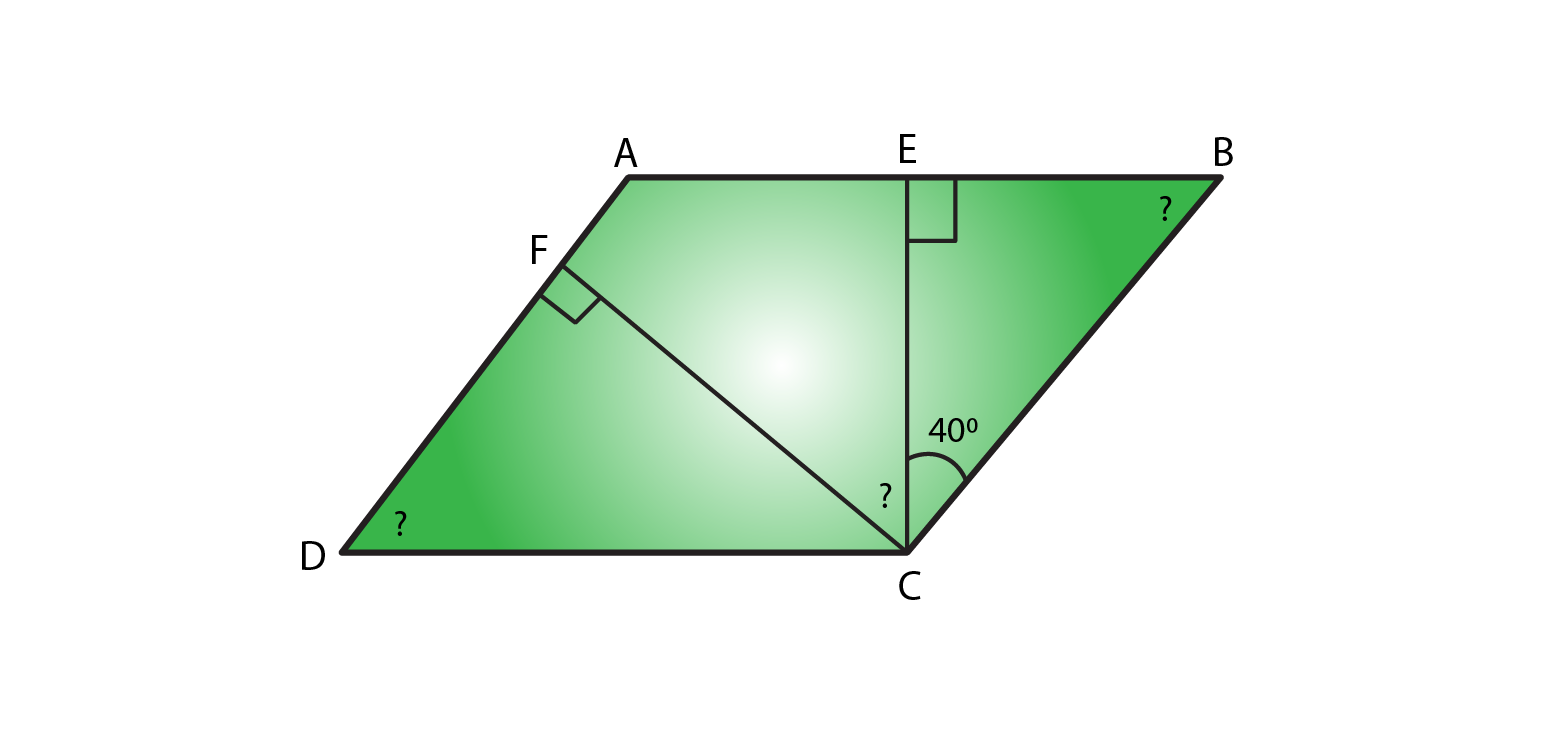Solution:

In ΔBEC

∠BEC + ∠ECB +∠CBE = 180° [Sum of angles of a triangle is 180°]

90° + 40° + ∠CBE = 180°

∠CBE = 180°-130°

∠CBE = 50°

∠CBE = ∠ADC = 50° (Opposite angles of a parallelogram are equal)

∠B = ∠D = 50° [Opposite angles of a parallelogram are equal]

∠A + ∠B = 180° [Sum of adjacent angles of a triangle is 180°]

∠A + 50° = 180°

∠A = 180°-50°

So, ∠A = 130°

In ΔDFC

∠DFC + ∠FCD +∠CDF = 180° [Sum of angles of a triangle is 180°]

90° + ∠FCD + 50° = 180°

∠FCD = 180°-140°

∠FCD = 40°

∠A = ∠C = 130° [Opposite angles of a parallelogram are equal]

∠C = ∠FCE +∠BCE + ∠FCD

∠FCD + 40° + 40° = 130°

∠FCD = 130° – 80°

∠FCD = 50°

∴ ∠EBC = 50o, ∠ADC = 50o and ∠FCD = 50o

21. The angle between the altitudes of a parallelogram, through the same vertex of an obtuse angle of the parallelogram is 60°. Find the angles of the parallelogram.

Solution: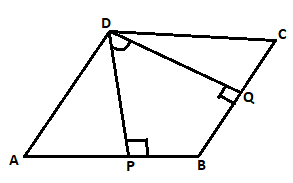Let us consider a parallelogram, ABCD. Where, DP⊥AB and DQ ⊥BC.

Given ∠PDQ = 60°

∠PDQ + ∠DPB + ∠B + ∠BQD = 360° [Sum of all the angles of a Quadrilateral is 360°]

60° + 90° + ∠B + 90° = 360°

∠B = 360° – 240°

∠B = 120°

∠B = ∠D = 120° [Opposite angles of parallelogram are equal]

∠B + ∠C = 180° [Sum of adjacent interior angles in a parallelogram is 180°]

120° + ∠C = 180°

∠C = 180° – 120° = 60°

∠A = ∠C = 60° (Opposite angles of parallelogram are equal)

∴ Angles of a parallelogram are 60o, 120o, 60o, 120o

22. In Figure, ABCD and AEFG are parallelograms. If ∠C =55°, what is the measure of ∠F?Solution:

In parallelogram ABCD

∠C = ∠A = 55° [In a parallelogram opposite angles are equal in a parallelogram]

In parallelogram AEFG

∠A = ∠F = 55° [In a parallelogram opposite angles are equal in a parallelogram]

∴ Measure of ∠F = 55°

23. In Figure, BDEF and DCEF are each a parallelogram. Is it true that BD = DC? Why or why not?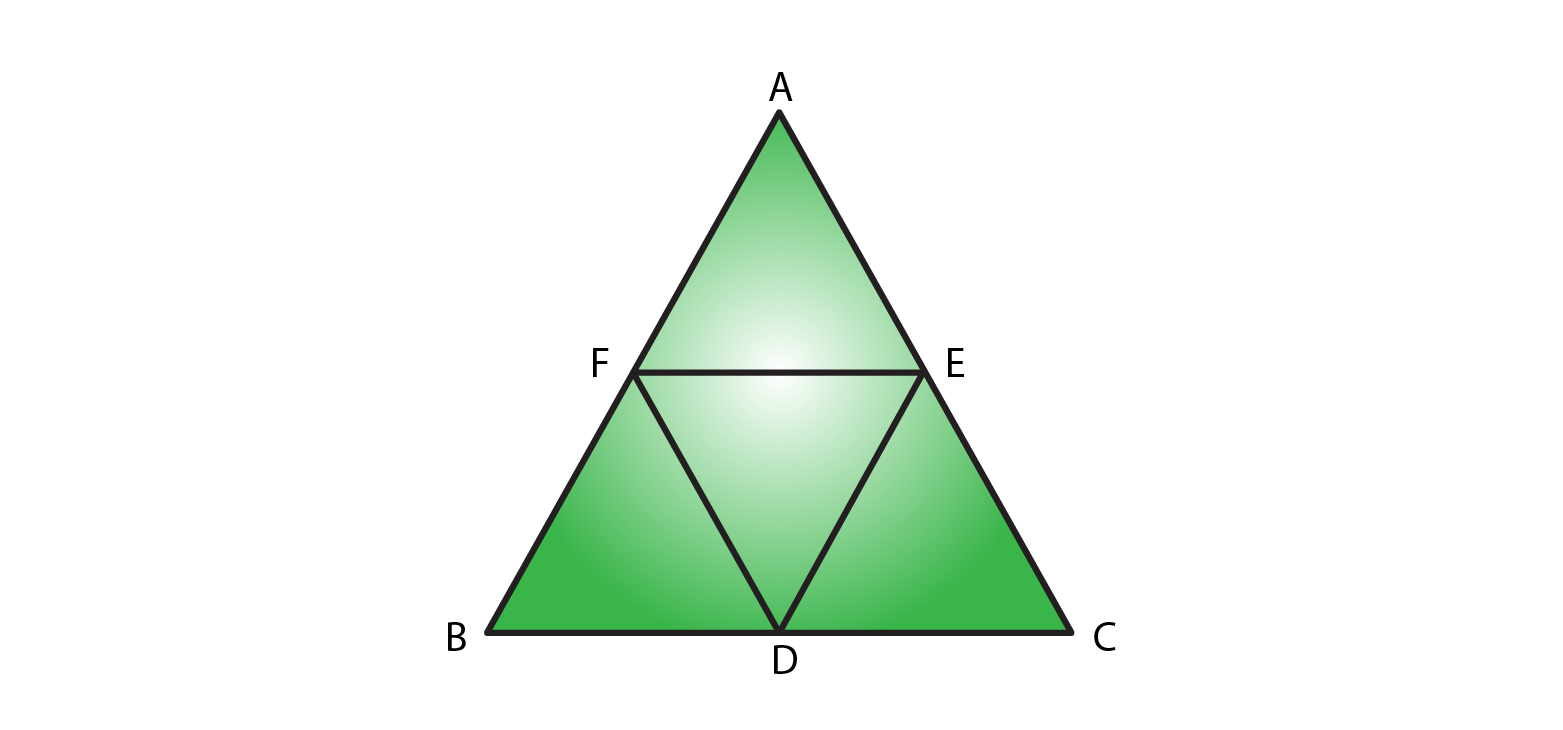Solution:

In parallelogram BDEF

BD = EF [In a parallelogram opposite sides are equal]

In parallelogram DCEF

DC = EF [In a parallelogram opposite sides are equal]

Since, BD = EF = DC

So, BD = DC

24. In Figure, suppose it is known that DE = DF. Then, is ΔABC isosceles? Why or why not?Solution:

In parallelogram BDEF

BD = EF and BF = DE [opposite sides are equal in a parallelogram]

In parallelogram DCEF

DC = EF and DF = CE [opposite sides are equal in a parallelogram]

In parallelogram AFDE

AF = DE and DF = AE [opposite sides are equal in a parallelogram]

So, DE = AF = BF

Similarly: DF = CE = AE

Given, DE = DF

Since, DF = DF

AF + BF = CE + AE

AB = AC

∴ ΔABC is an isosceles triangle.

25. Diagonals of parallelogram ABCD intersect at O as shown in Figure. XY contains O, and X, Y are points on opposite sides of the parallelogram. Give reasons for each of the following:

(i) OB = OD

(ii) ∠OBY = ∠ODX

(iii) ∠BOY = ∠DOX

(iv) ΔBOY = ΔDOX

Now, state if XY is bisected at O.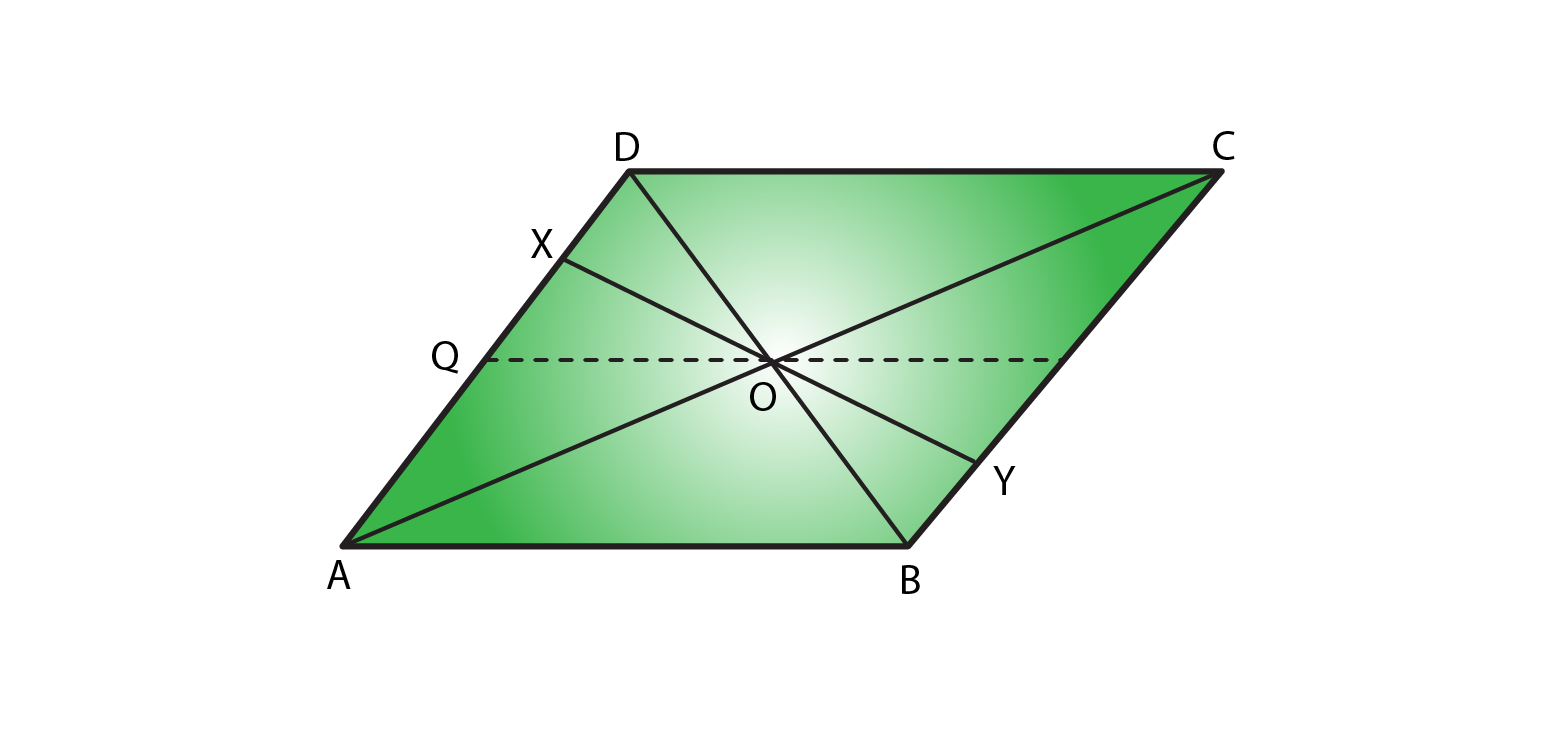Solution:

(i) OB = OD

OB = OD. Since diagonals bisect each other in a parallelogram.

(ii) ∠OBY =∠ODX

∠OBY =∠ODX. Since alternate interior angles are equal in a parallelogram.

(iii) ∠BOY= ∠DOX

∠BOY= ∠DOX. Since vertical opposite angles are equal in a parallelogram.

(iv) ΔBOY ≅ ΔDOX

ΔBOY and ΔDOX. Since OB = OD, where diagonals bisect each other in a parallelogram.

∠OBY =∠ODX [Alternate interior angles are equal]

∠BOY= ∠DOX [Vertically opposite angles are equal]

ΔBOY ≅ΔDOX [by ASA congruence rule]

OX = OY [Corresponding parts of congruent triangles]

∴ XY is bisected at O.

26. In Fig. 17.31, ABCD is a parallelogram, CE bisects ∠C and AF bisects ∠A. In each of the following, if the statement is true, give a reason for the same:

(i) ∠A = ∠C

(ii) ∠FAB = ½ ∠A

(iii) ∠DCE = ½ ∠C

(iv) ∠CEB = ∠FAB

(v) CE ∥ AF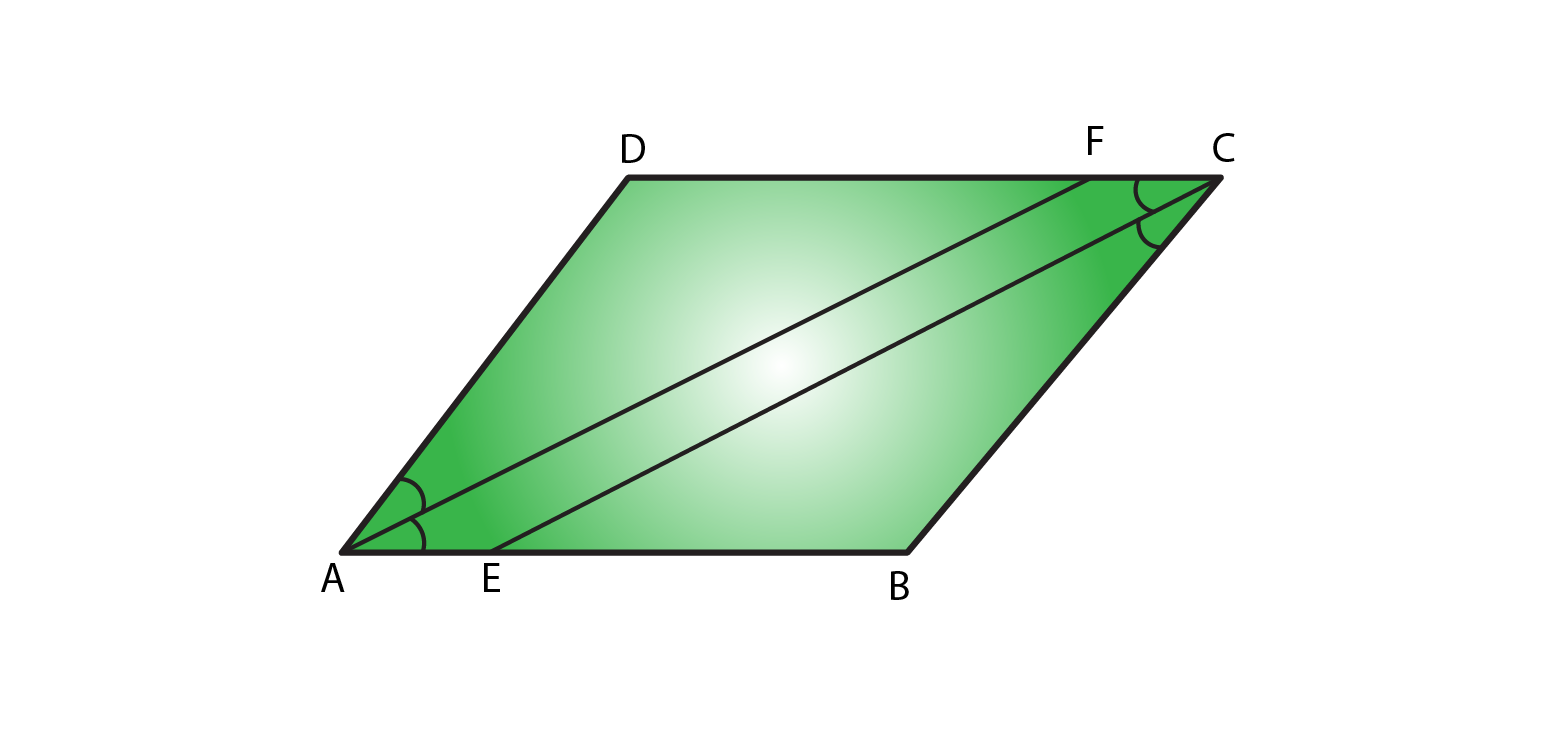Solution:

(i) ∠A = ∠C

True, Since ∠A =∠C = 55° [opposite angles are equal in a parallelogram]

(ii) ∠FAB = ½ ∠A

True, Since AF is the angle bisector of ∠A.

(iii) ∠DCE= ½ ∠C

True, Since CE is the angle bisector of angle ∠C.

(iv) ∠CEB= ∠FAB

True,

Since ∠DCE = ∠FAB (opposite angles are equal in a parallelogram).

∠CEB = ∠DCE (alternate angles)

½ ∠C = ½ ∠A [AF and CE are angle bisectors]

(v) CE || AF

True, since one pair of opposite angles are equal, therefore quad. AEFC is a parallelogram.

27. Diagonals of a parallelogram ABCD intersect at O. AL and CM are drawn perpendiculars to BD such that L and M lie on BD. Is AL = CM? Why or why not?

Solution: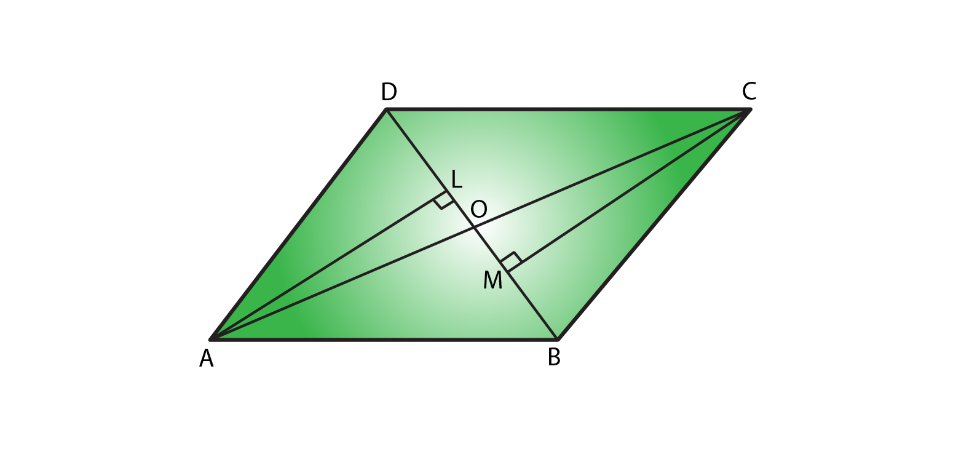Given, AL and CM are perpendiculars on diagonal BD.

In ΔAOL and ΔCOM

∠AOL = ∠COM (vertically opposite angle) ….. (i)

∠ALO = ∠CMO = 90o (each right angle) ……. (ii)

By using angle sum property

∠AOL + ∠ALO + ∠LAO = 180o ……… (iii)

∠COM + ∠CMO + ∠OCM = 180o ……. (iv)

From (iii) and (iv)

∠AOL + ∠ALO + ∠LAO = ∠COM + ∠CMO + ∠OCM

∠LAO = ∠OCM (from (i) and (ii))

In ΔAOL and ΔCOM

∠ALO = ∠CMO (each right angle)

AO = OC (diagonals of a parallelogram bisect each other)

∠LAO = ∠OCM (proved)

So, ΔAOL is congruent to ΔCOM

∴ AL = CM (Corresponding parts of congruent triangles)

28. Points E and F lie on diagonals AC of a parallelogram ABCD such that AE = CF. what type of quadrilateral is BFDE?

Solution: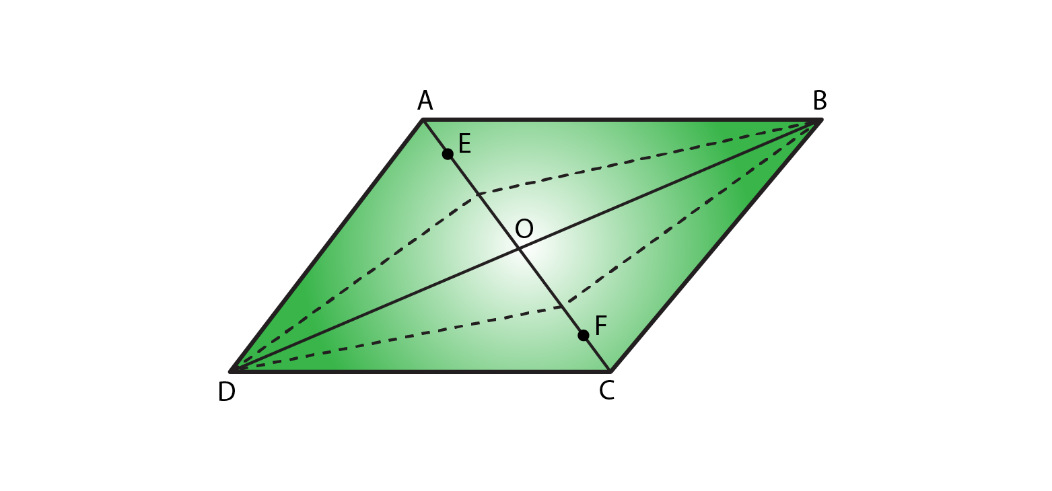In parallelogram ABCD:

AO = OC…………….. (i) (Diagonals of a parallelogram bisect each other)

AE = CF…….. (ii) Given

On subtracting (ii) from (i)

AO – AE = OC – CF

EO = OF ….. (iii)

In ΔDOE and ΔBOF

EO = OF (proved)

DO = OB (Diagonals of a parallelogram bisect each other)

∠DOE = ∠BOF (vertically opposite angles are equal in a parallelogram)

By the rule of SAS congruence ΔDOE ≅ ΔBOF

So, DE = BF (Corresponding parts of congruent triangles)

In ΔBOE and ΔDOF

EO = OF (proved)

DO = OB (diagonals of a parallelogram bisect each other)

∠DOF = ∠BOE (vertically opposite angles are equal in a parallelogram)

By the rule of SAS congruence ΔDOE ≅ ΔBOF

∴ DF = BE (Corresponding parts of congruent triangles)

∴ BFDE is a parallelogram, since one pair of opposite sides are equal and parallel.

29. In a parallelogram ABCD, AB = 10cm, AD = 6 cm. The bisector of ∠A meets DC in E, AE and BC produced meet at F. Find the length CF.

Solution: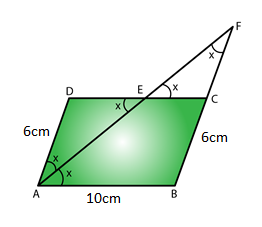In a parallelogram ABCD

Given, AB = 10 cm, AD = 6 cm

⇒ CD = AB = 10 cm and AD = BC = 6 cm [In a parallelogram opposite sides are equal]

AE is the bisector of ∠DAE = ∠BAE = x

∠BAE = ∠AED = x (alternate angles are equal)

ΔADE is an isosceles triangle. Since opposite angles in ΔADE are equal.

AD = DE = 6cm (opposite sides are equal)

CD = DE + EC

EC = CD – DE

= 10 – 6

= 4cm

∠DEA = ∠CEF = x (vertically opposite angle are equal)

∠EAD = ∠EFC = x (alternate angles are equal)

ΔEFC is an isosceles triangle. Since opposite angles in ΔEFC are equal.

CF = CE = 4cm (opposite side are equal to angles)

∴ CF = 4cm.